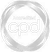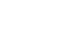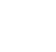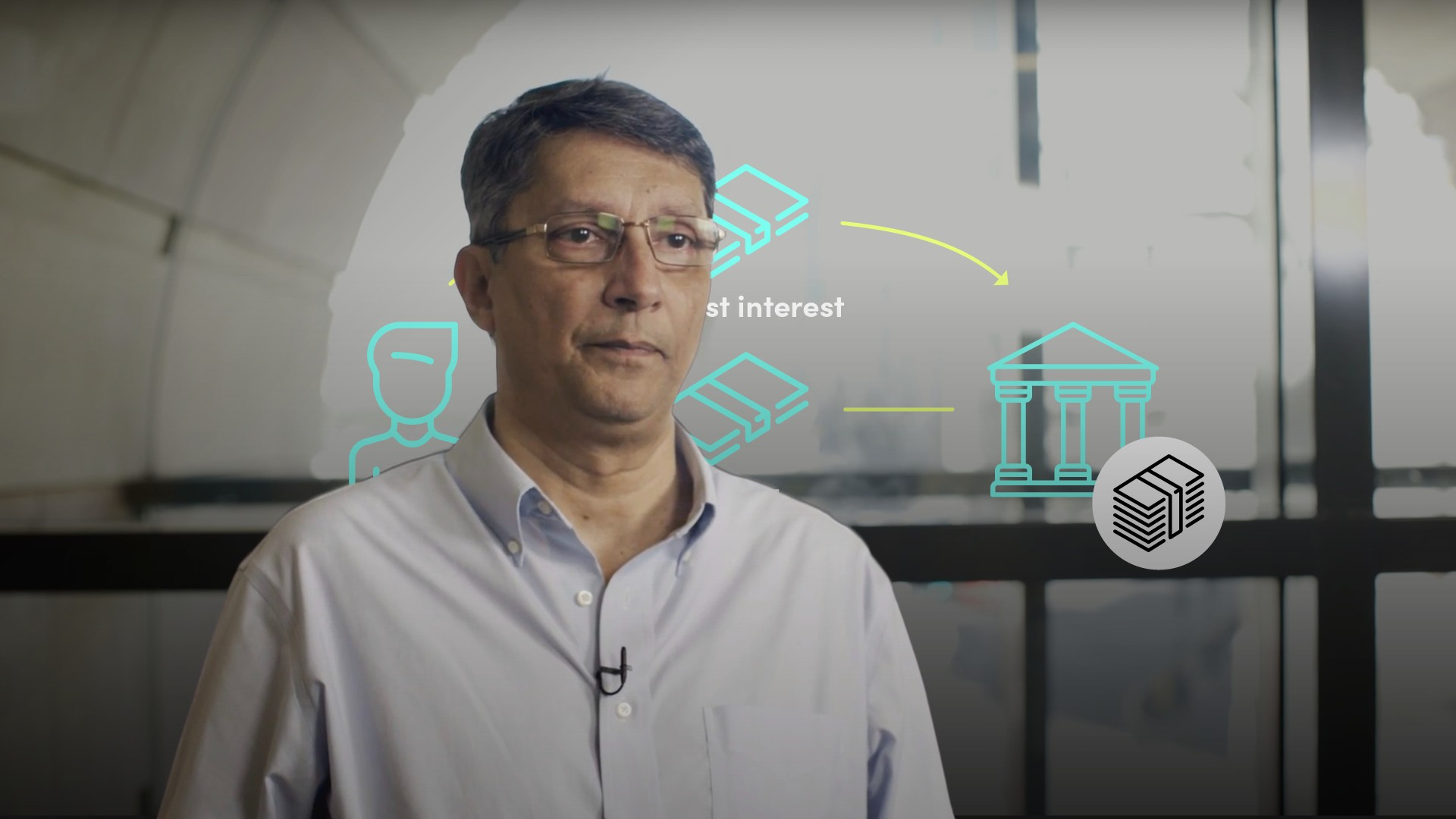# Simple vs Compound Interest### Abdulla Javeri

Compounding is one of the most powerful concepts in finance. Abdulla compares simple versus compound interest and the importance of understanding the difference.

Financial MathsCompounding is one of the most powerful concepts in finance. Abdulla compares simple versus compound interest and the importance of understanding the difference.

Finance Unlocked is the video learning platform built for finance professionals.### Simple vs Compound Interest

5 mins 28 secs

Overview

With simple interest, a depositor receiving annual interest from a deposit removes the interest and just reinvests the principal and earns the annual interest rate. With compound interest, the depositor reinvests the principal and interest every year i.e. the interest earns interest so the amount of interest received goes up each year.

Key learning objectives:

• Apply the simple vs compound interest rate scenario to an investment

• Learn how simple and compound interest differ

## Book a demo to access

Summary

#### How do simple and compound interest differ?

With simple interest, a depositor receiving annual interest removes the interest and just reinvests the principal, hence earns the annual interest rate. With compound interest, the depositor reinvests the principal and interest every year - the interest earns interest so the amount received goes up each year. Simple interest of 5% earned on \$1,000 over 50 years equals \$2,500, giving a total value of \$3,500. With compounding, the total value at the end of the period is \$11,467. The higher the interest rate the bigger the difference (albeit the same in percentage terms).

#### Apply the simple vs compound interest rate scenario to an investment

Investing \$1,000 in equities, removing the annual dividend and earning the capital gain has the same effect from a returns perspective as earning simple interest. Reinvesting the annual dividend by buying more equity has the same effect as interest compounding. Assuming an annual capital gain of 10% and a dividend yield of 3.5% over 50 years but not reinvesting the dividend results in a portfolio value of \$117,391 for a capital gain of \$116,391 (final value minus the initial \$1,000 investment) plus dividends of \$40,737 giving a total gain in capital and dividends of \$157,128. If dividends were reinvested, the capital value of the portfolio at the end of 50 years is \$521,101. Reinvesting the dividend makes up a substantial part of the return.

## Book a demo to access

Expert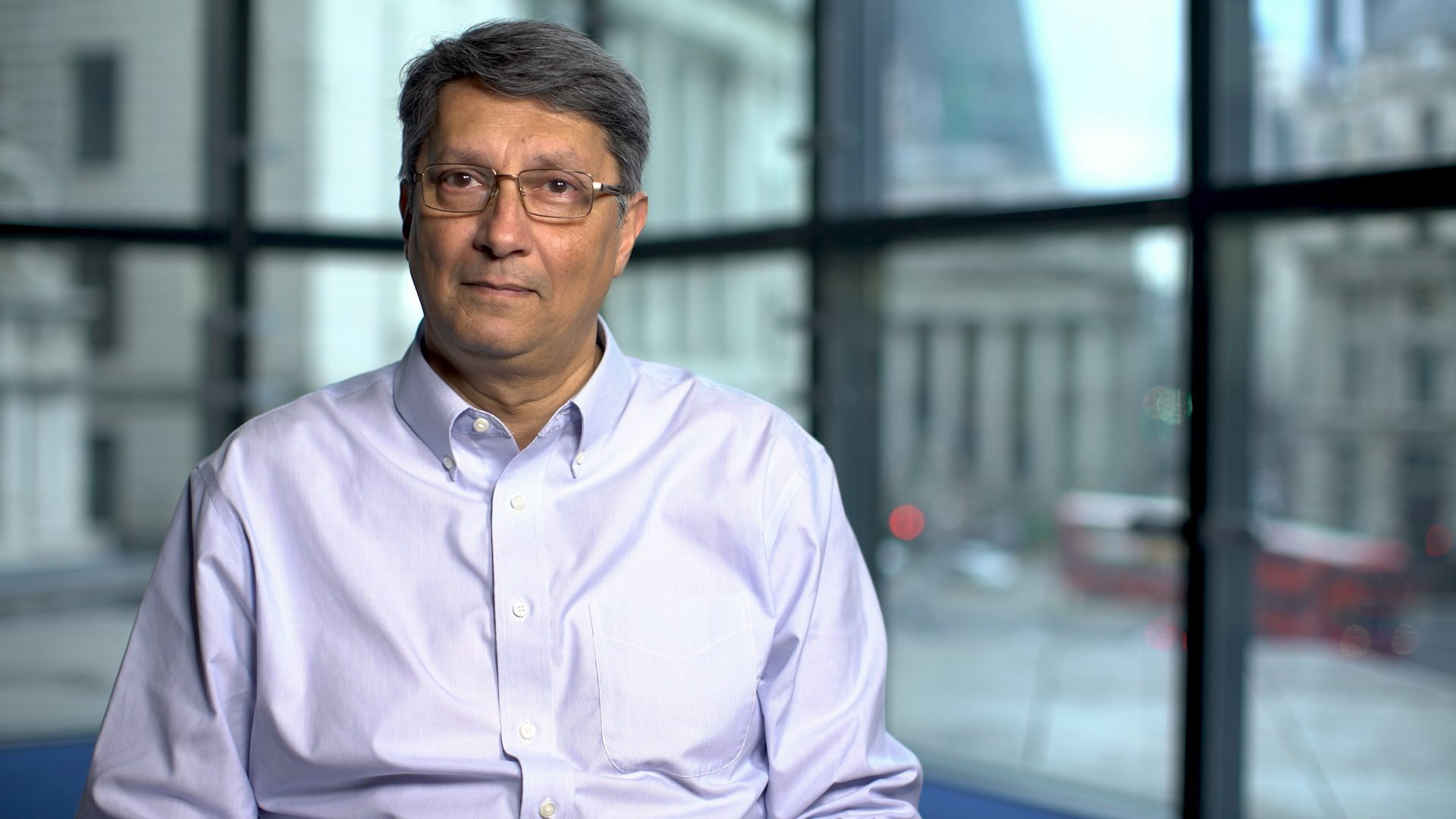### Abdulla Javeri

Abdulla’s career in the financial markets started in 1990 when he entered the trading floor of the London International Financial Futures Exchange, LIFFE, and qualified as a pit trader in equity and equity index options. In 1996, Abdulla became a trainer for regulatory qualifications and then for non-exam courses, primarily covering all major financial products.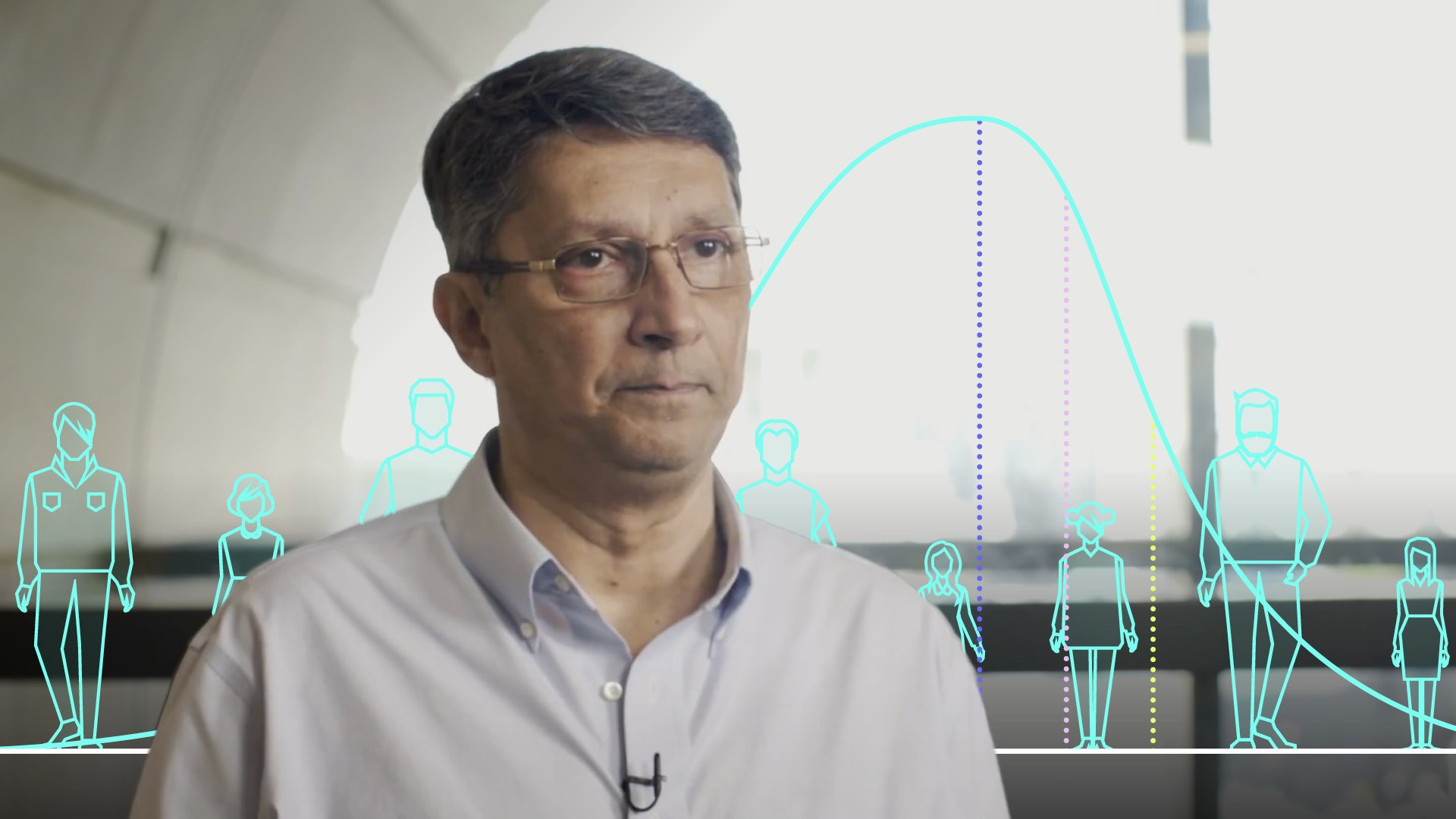03:18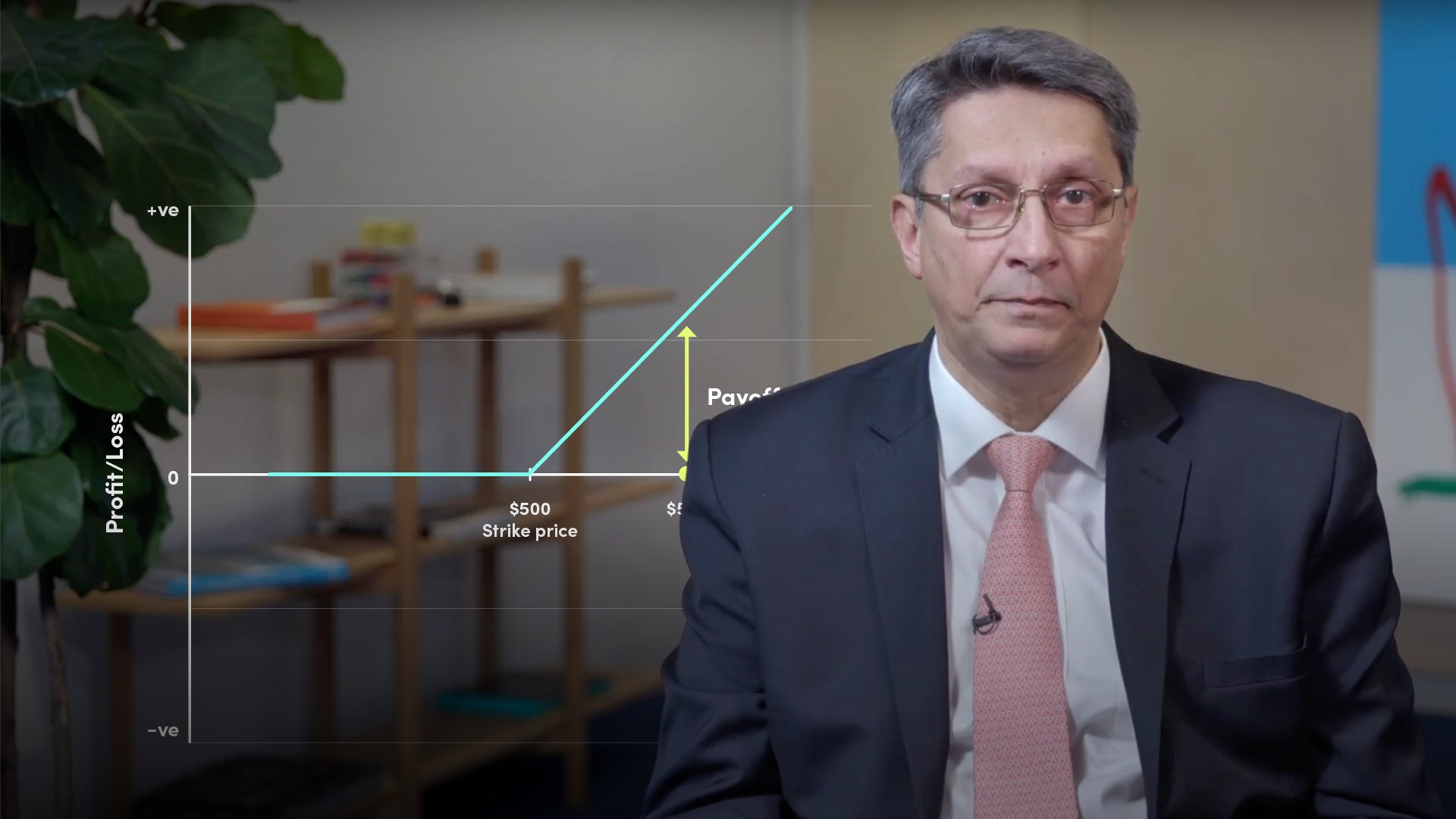05:12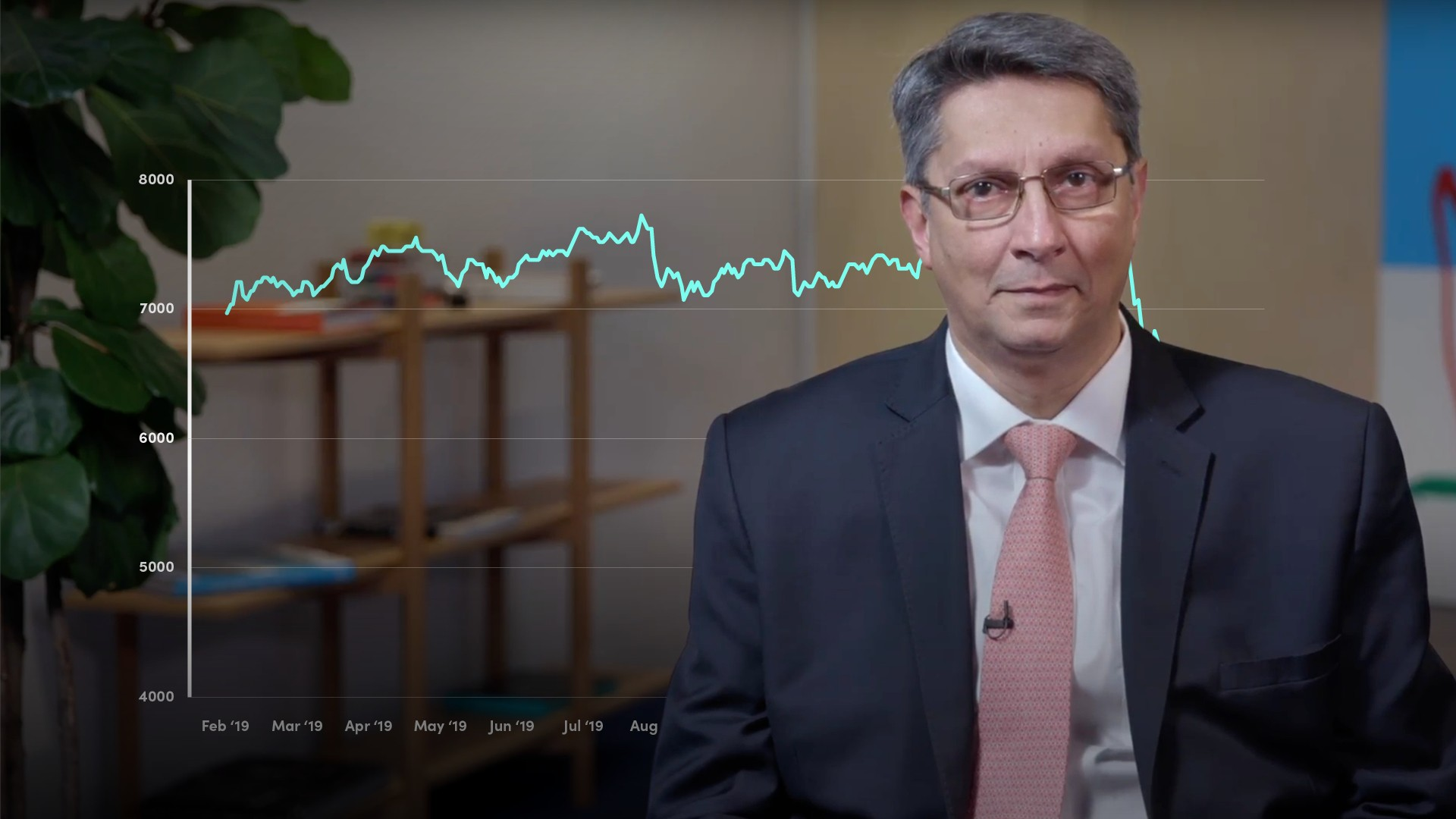05:39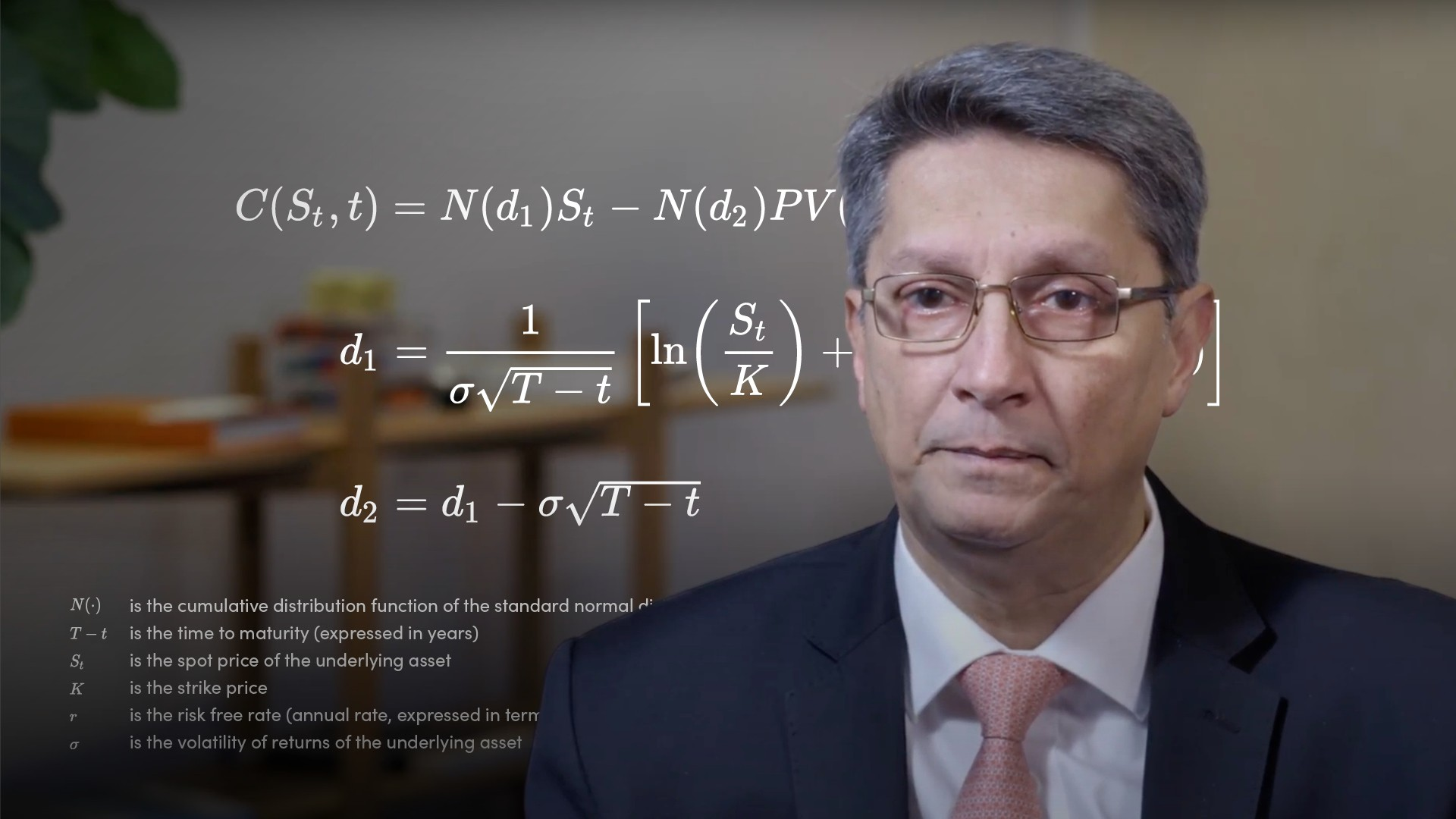05:08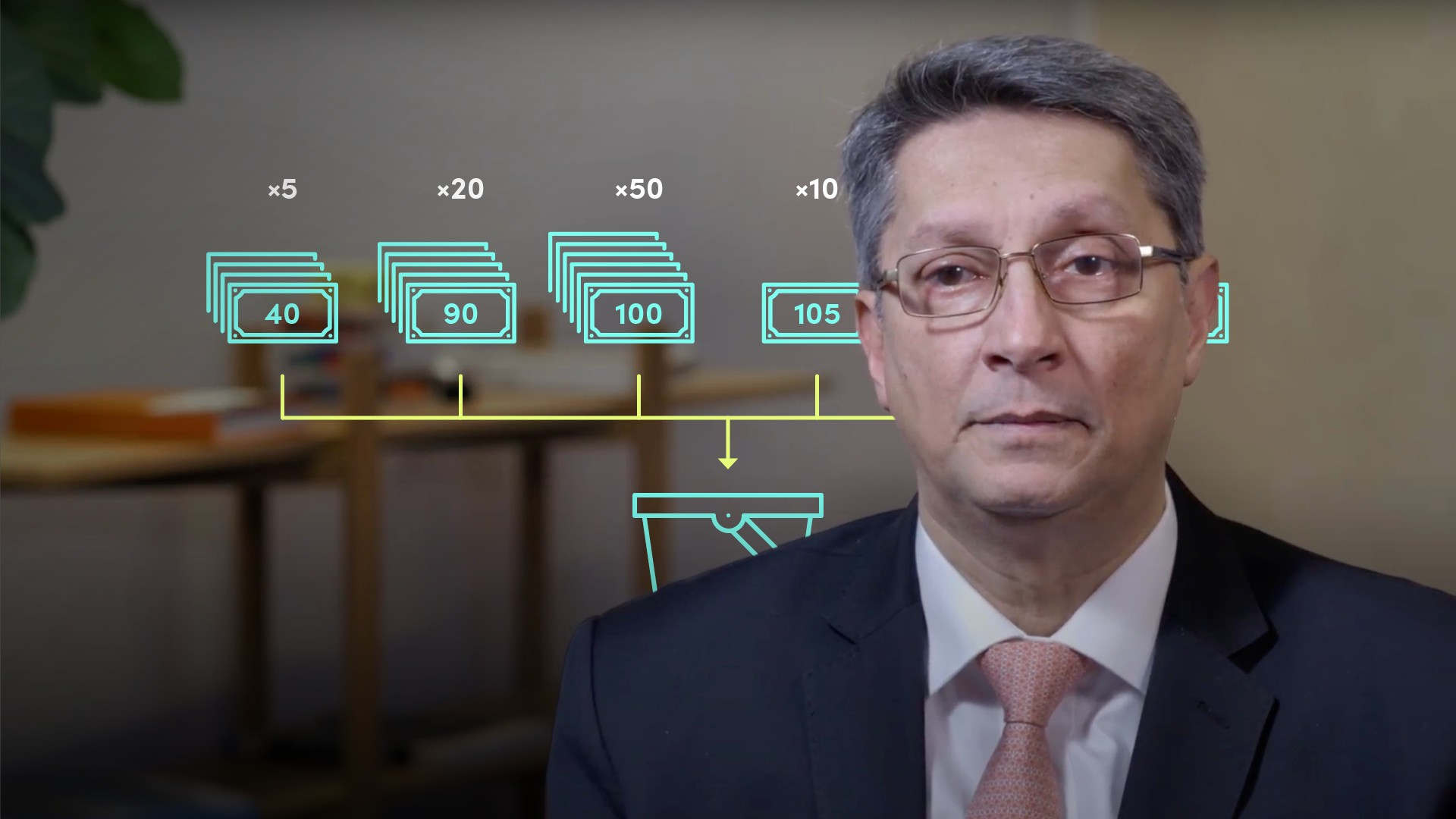04:52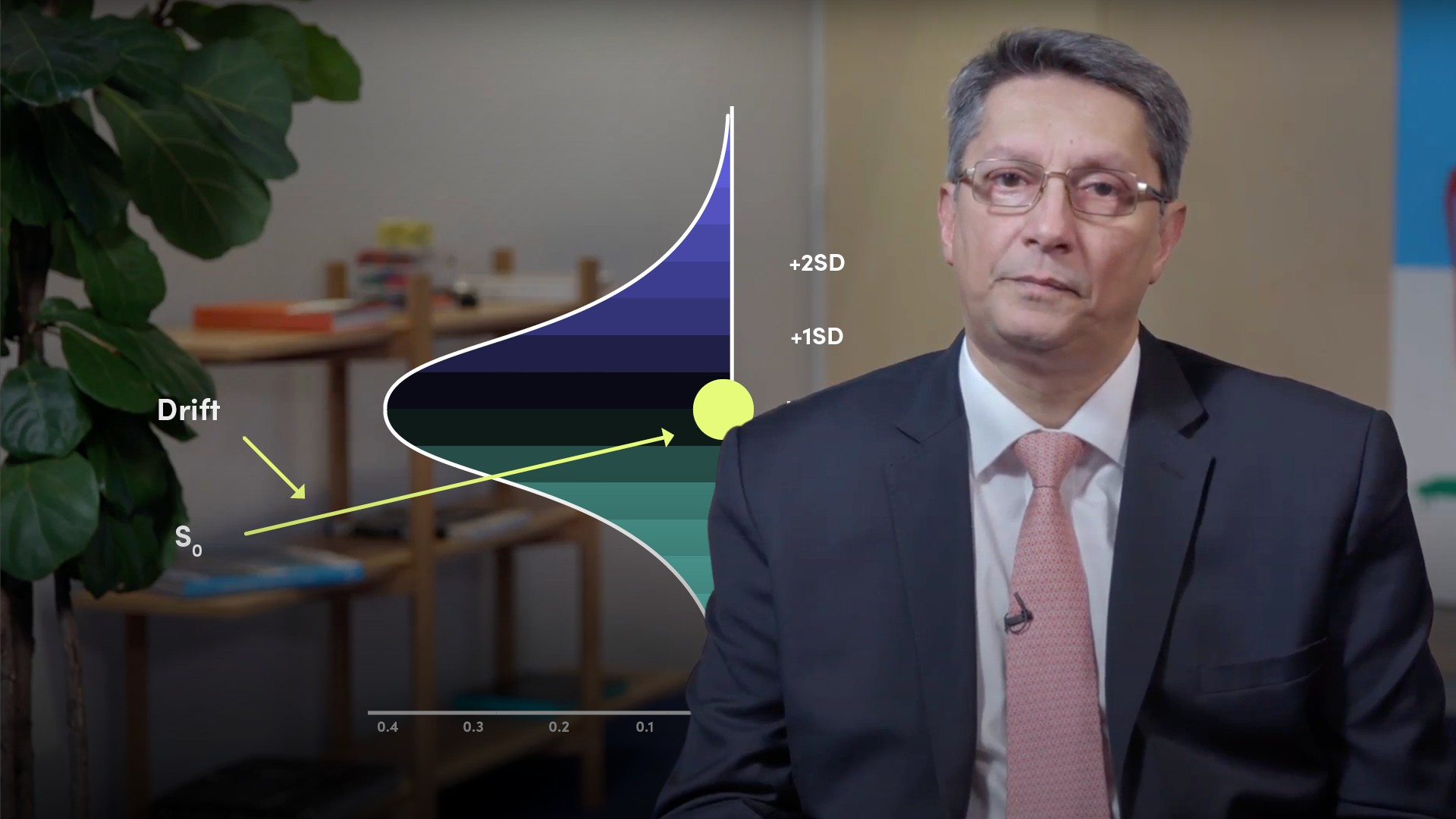05:09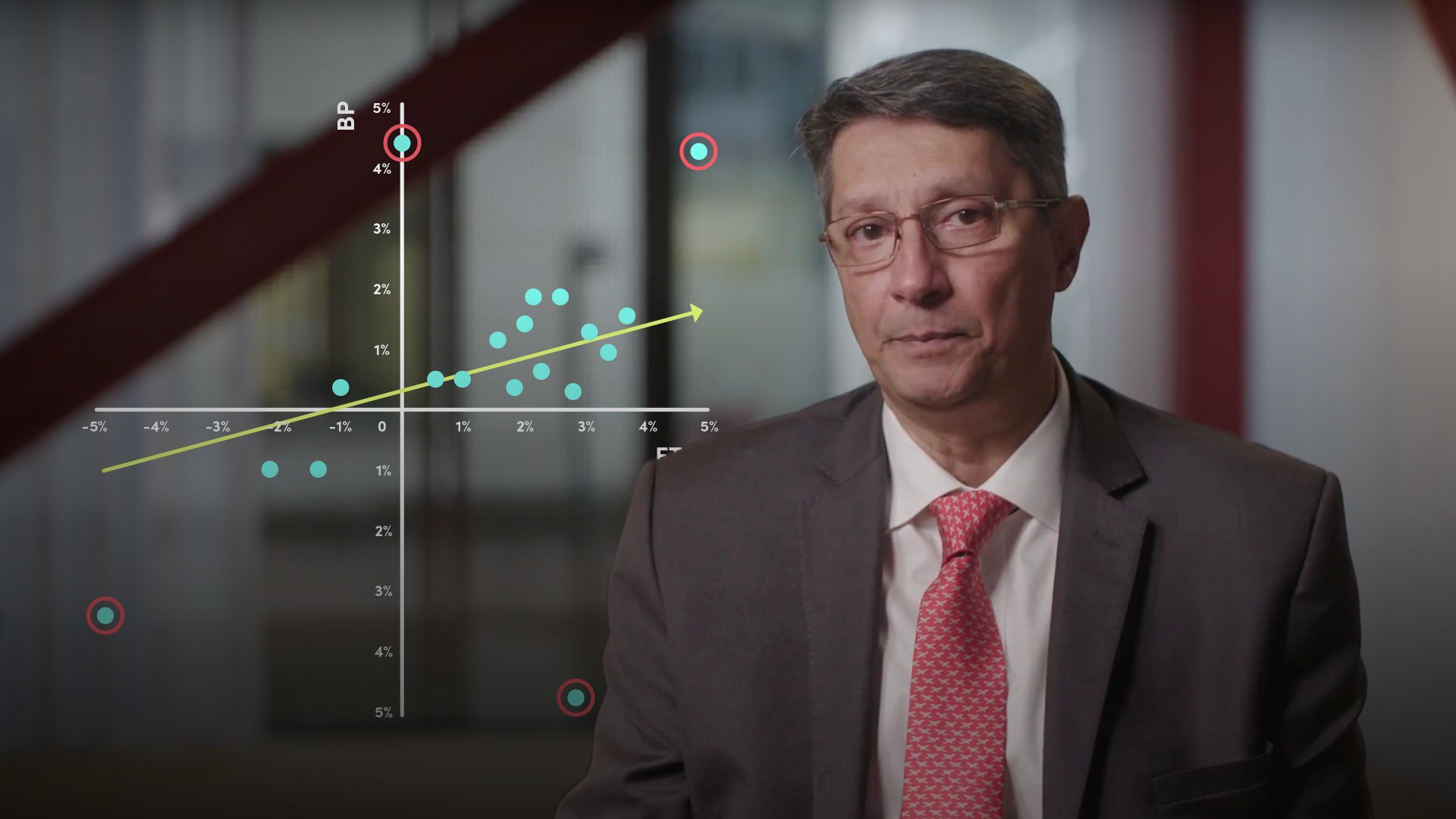04:13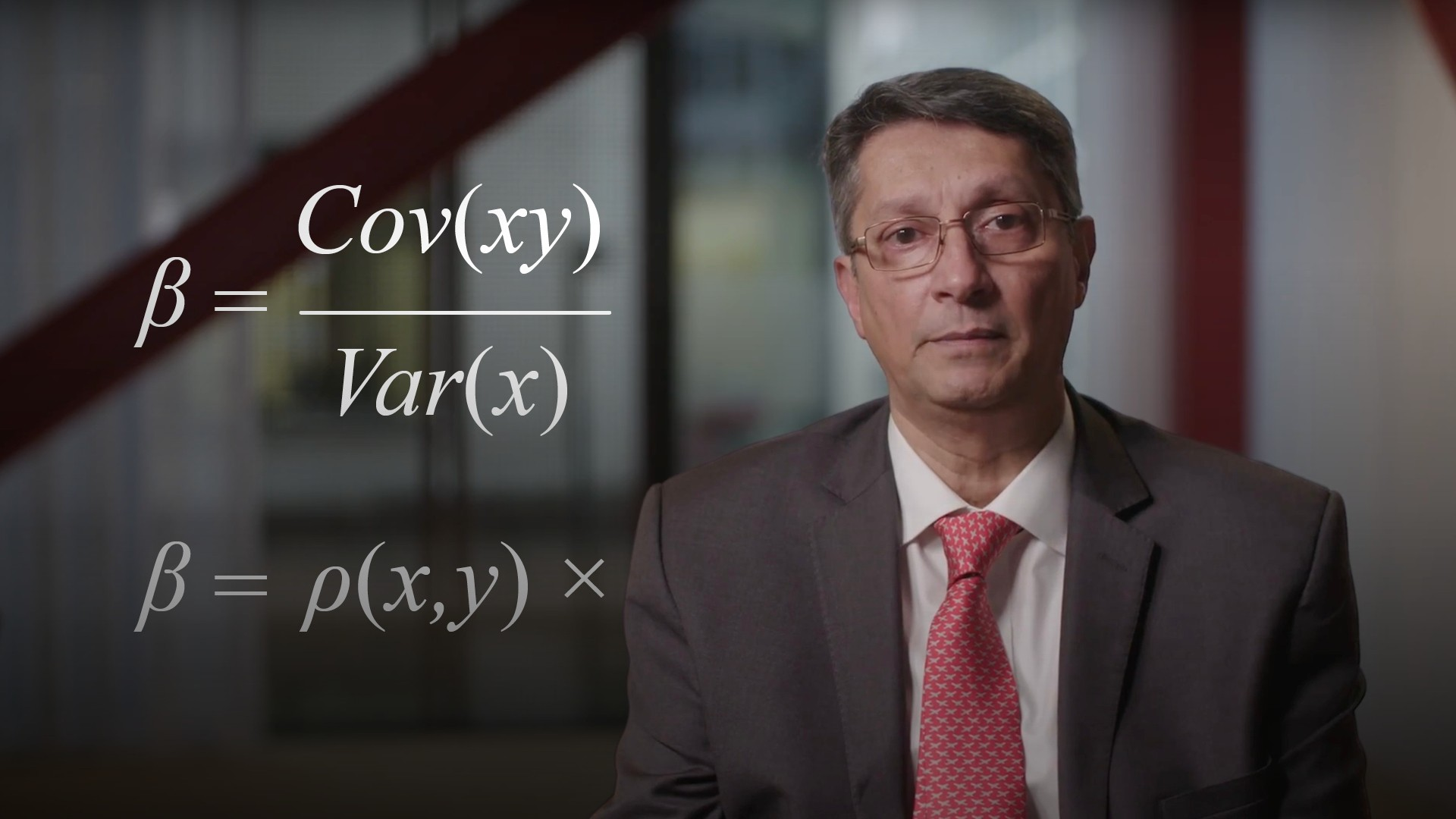05:3804:08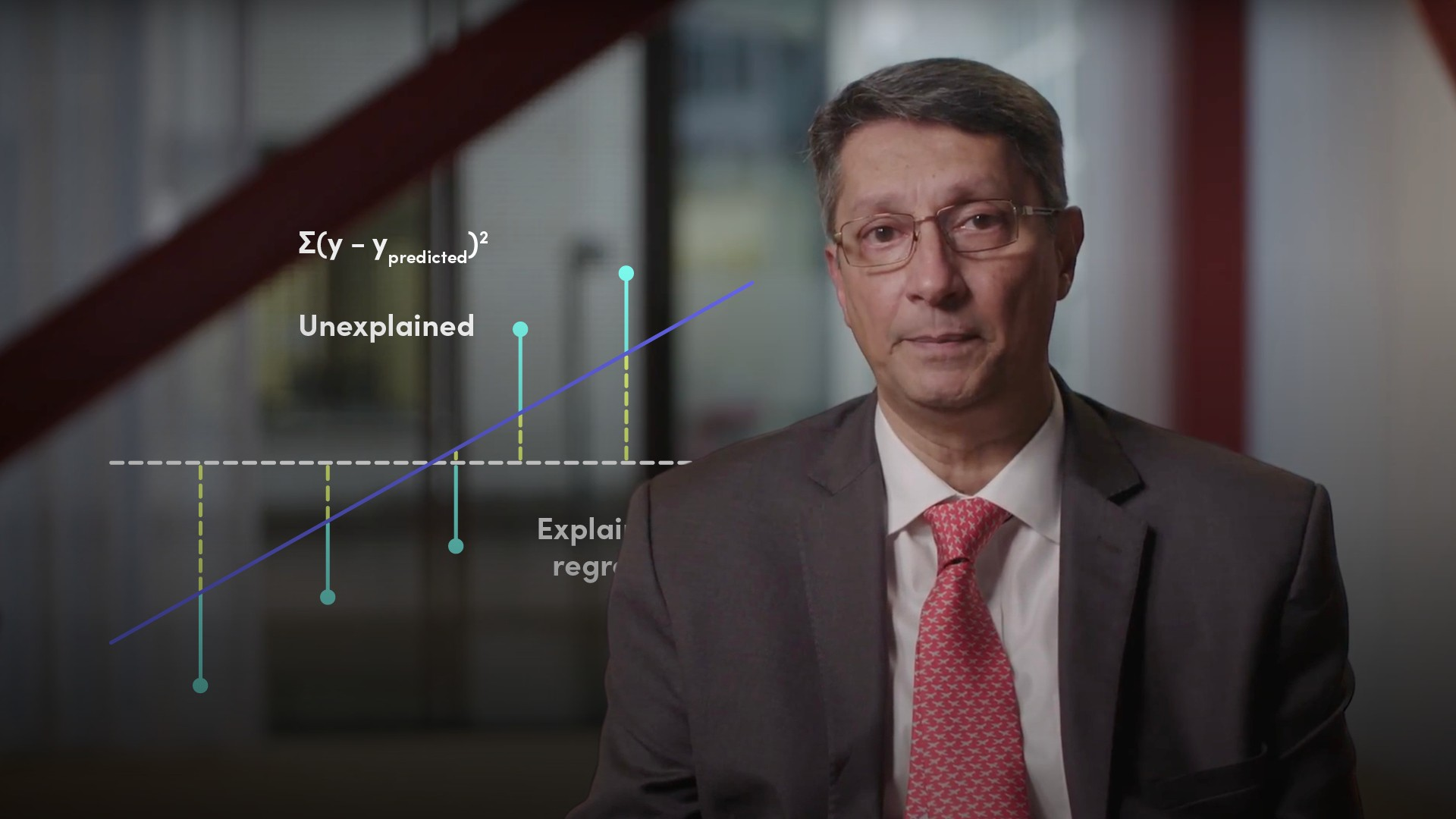04:22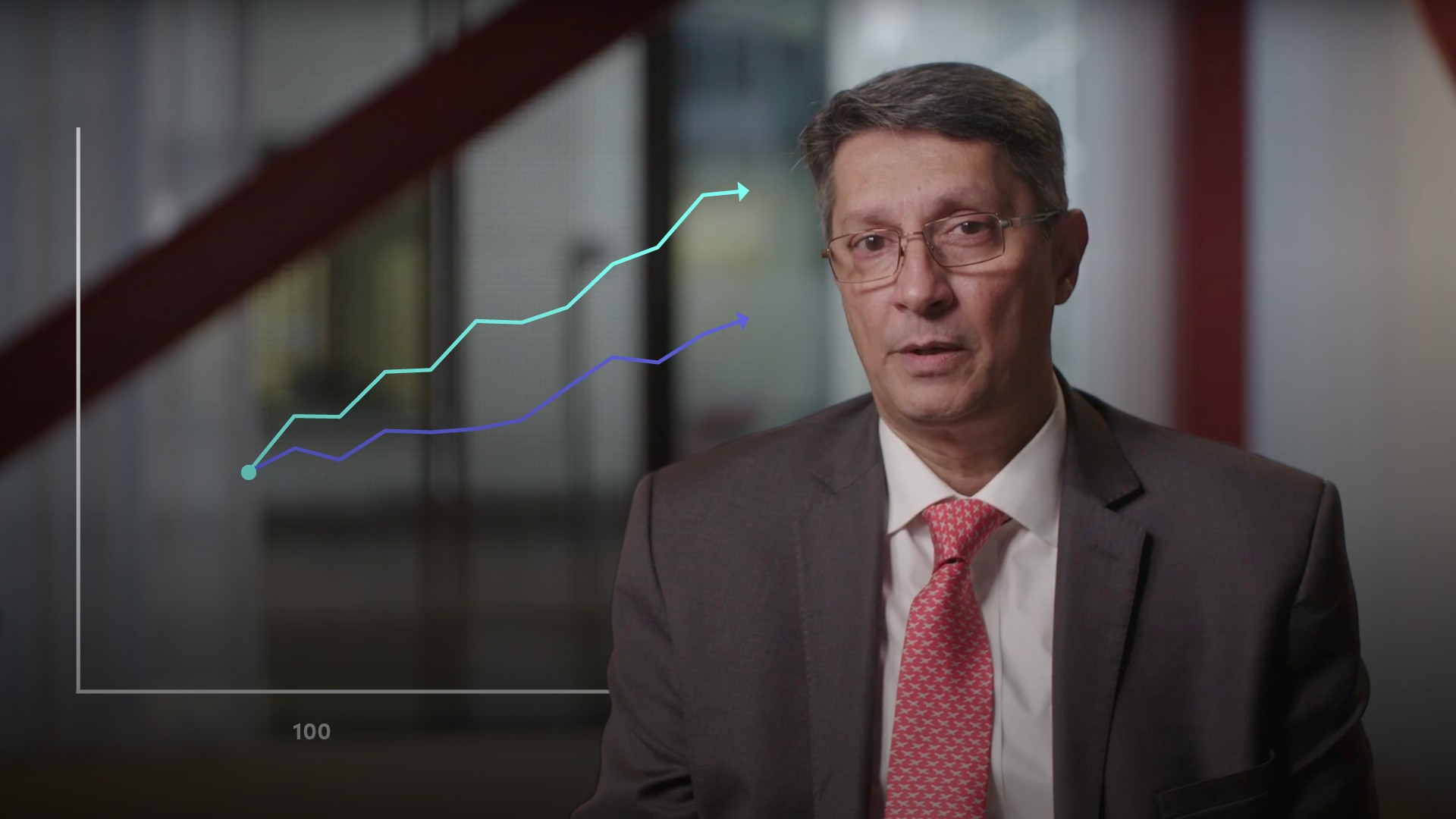05:02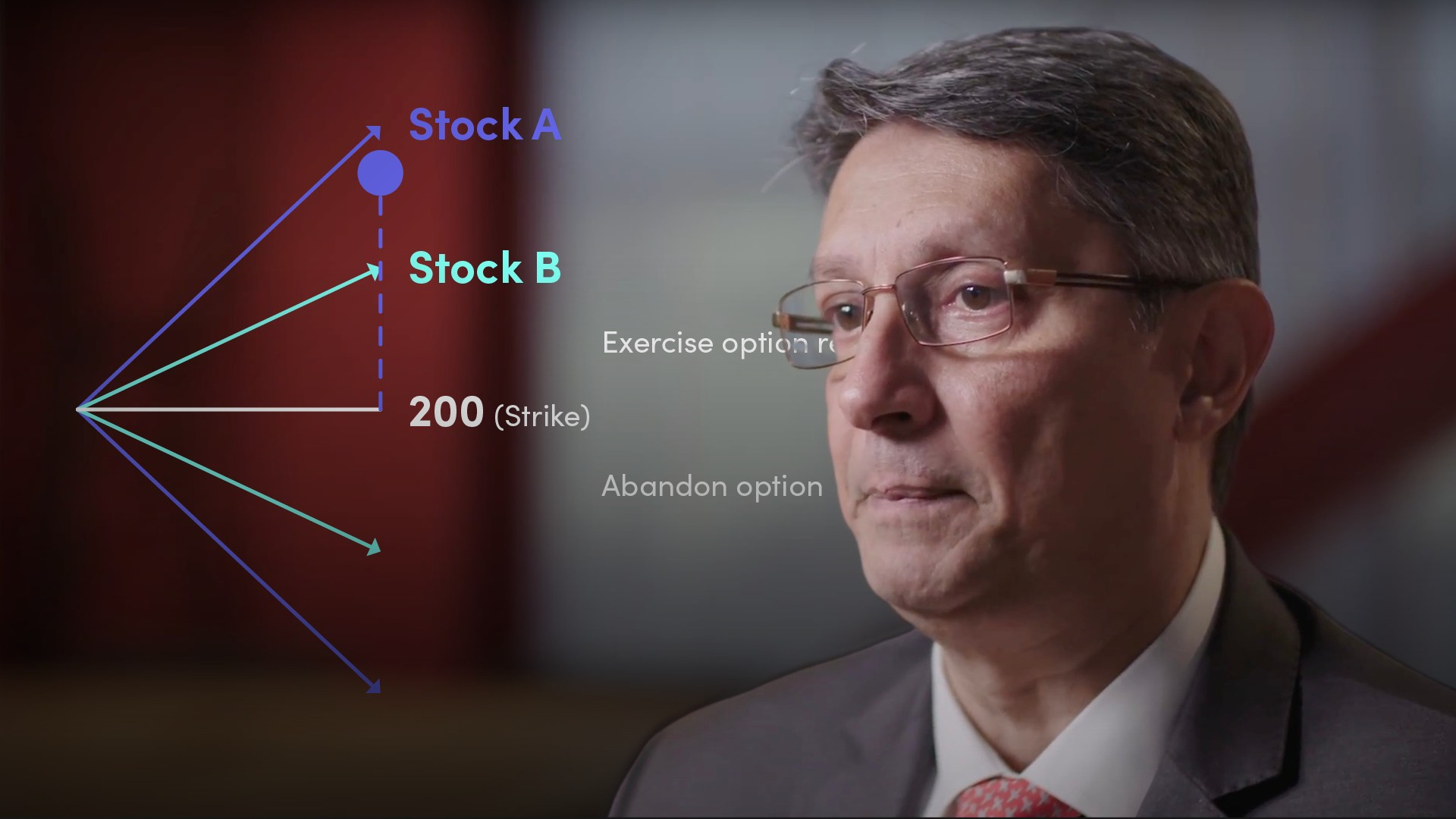03:26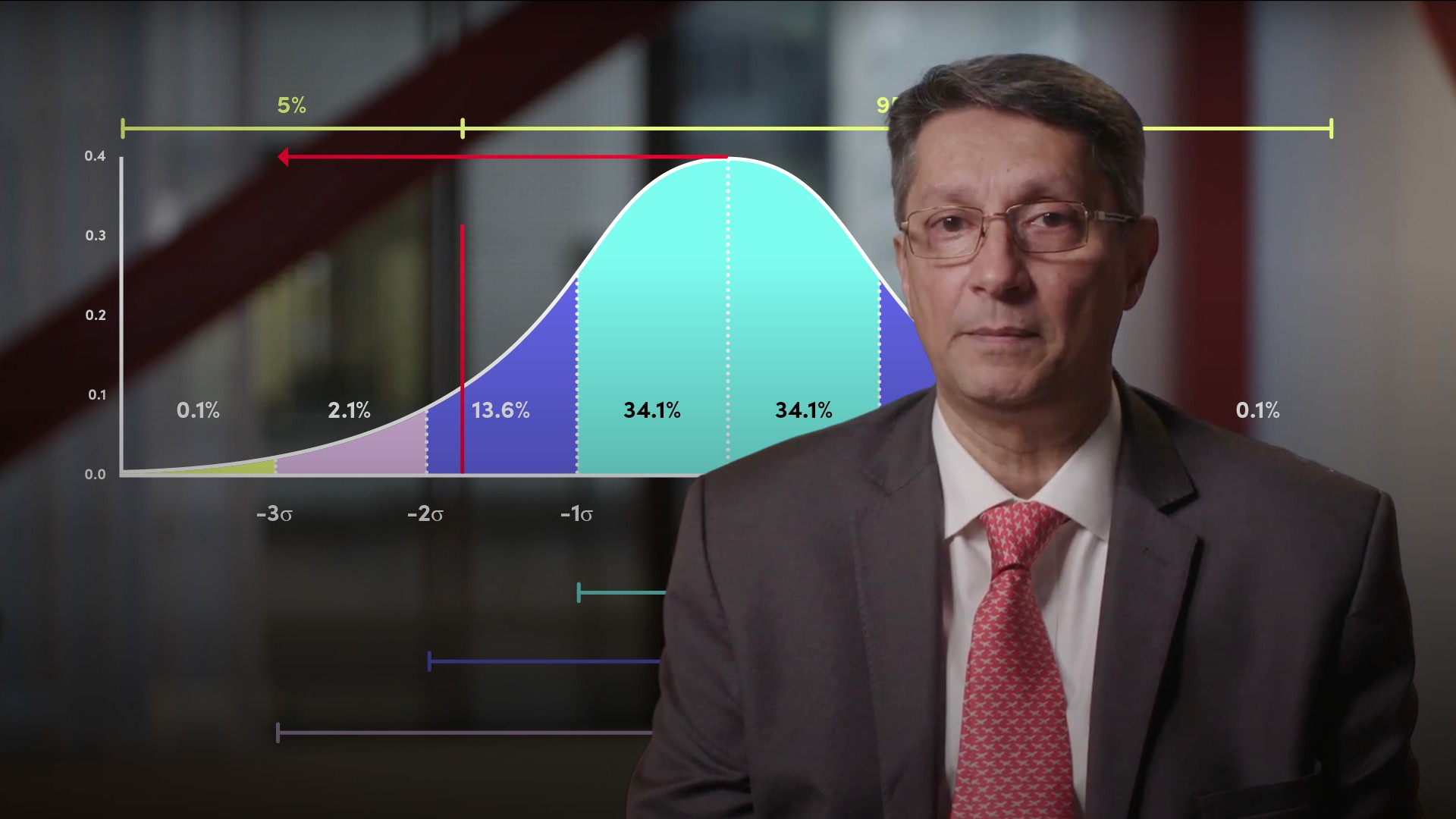03:58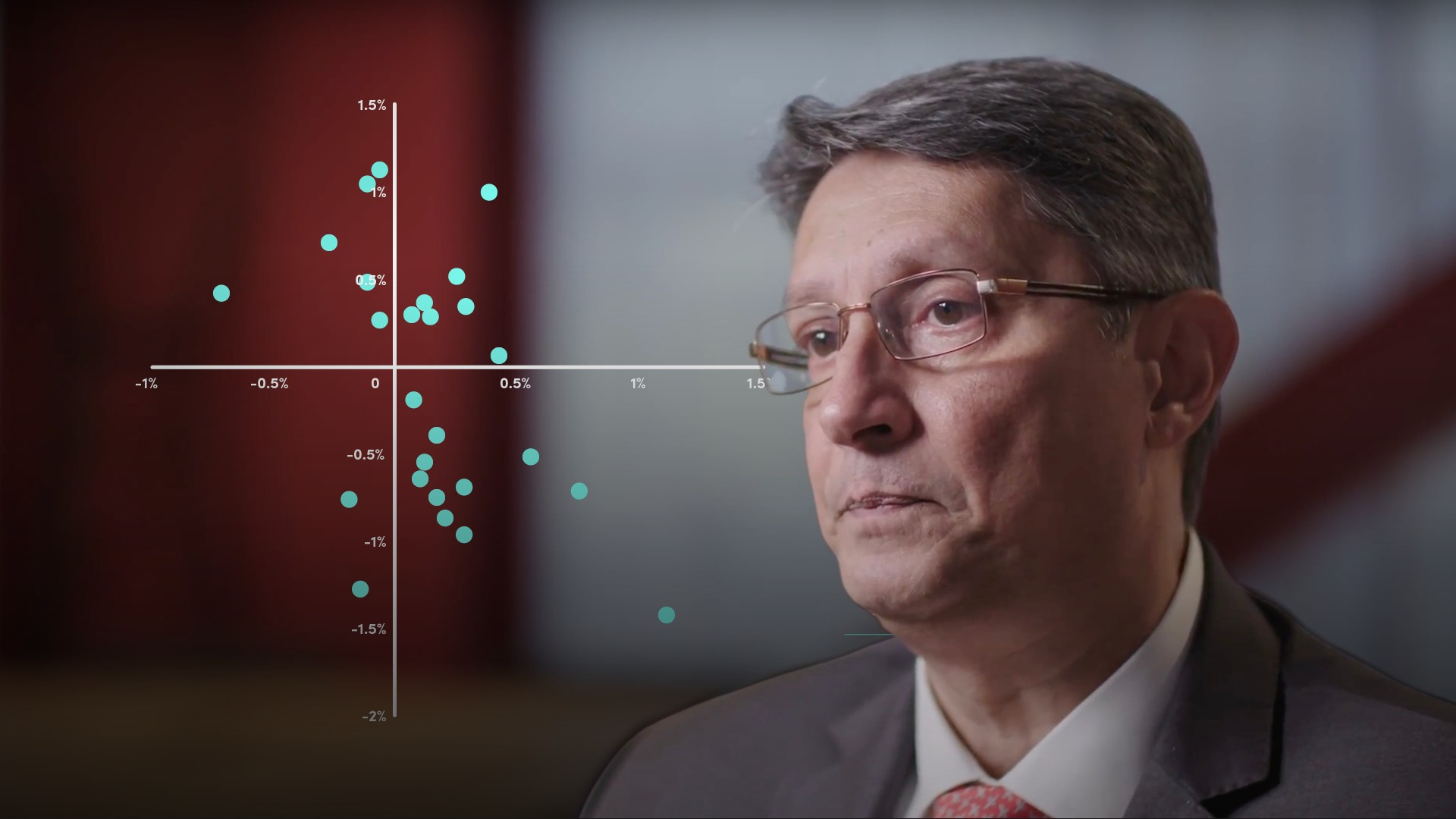04:55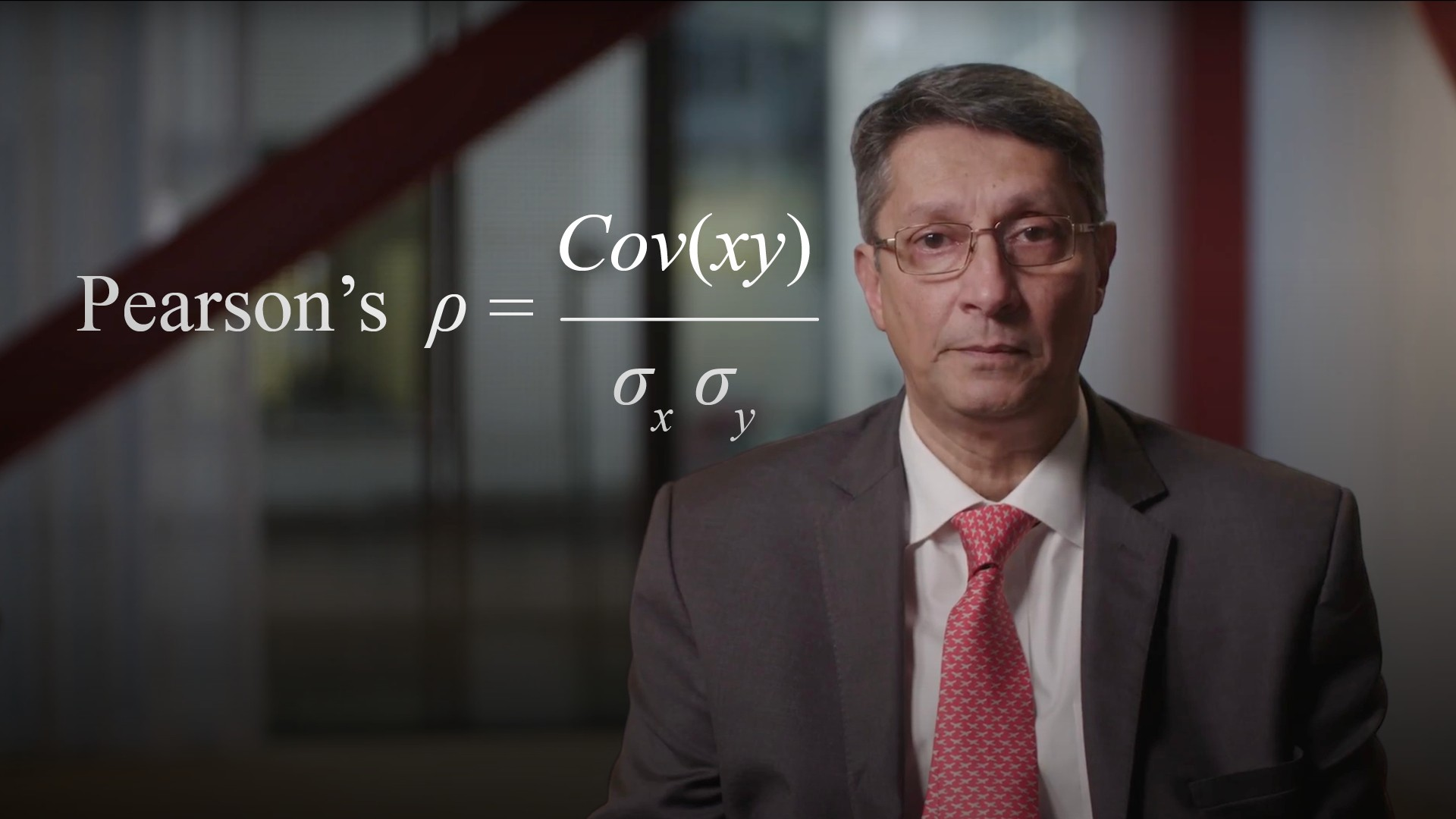04:56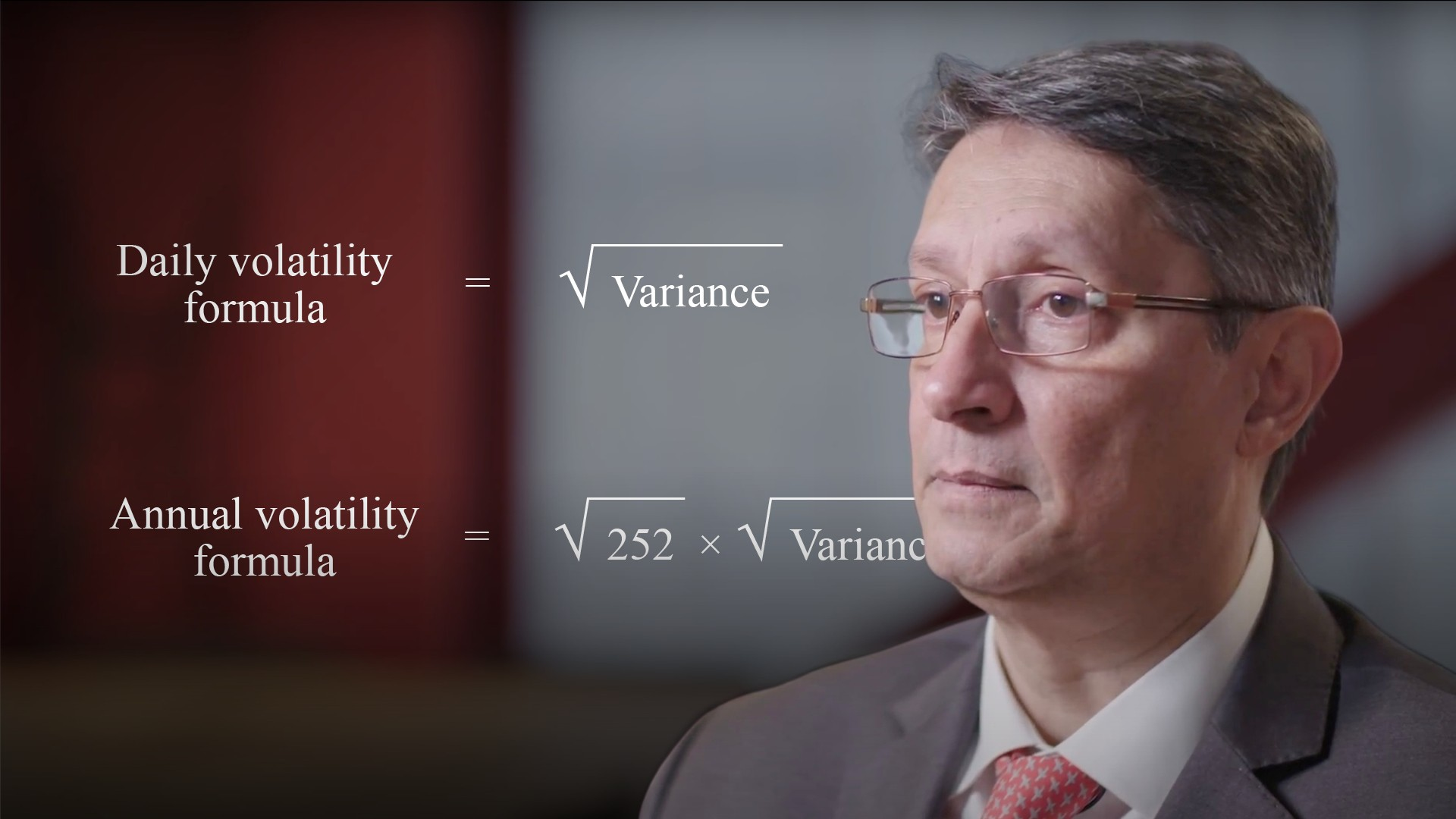04:34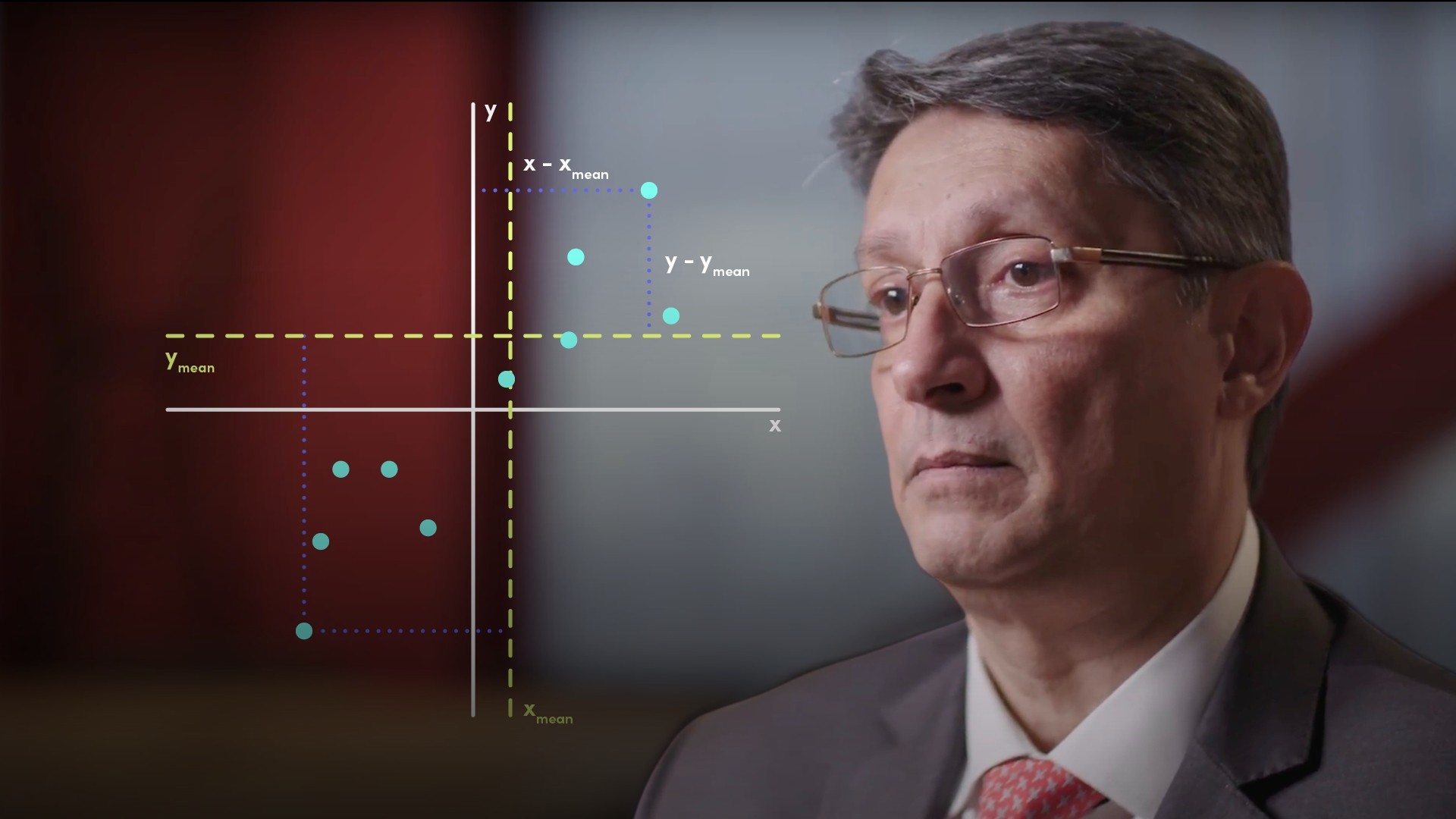04:40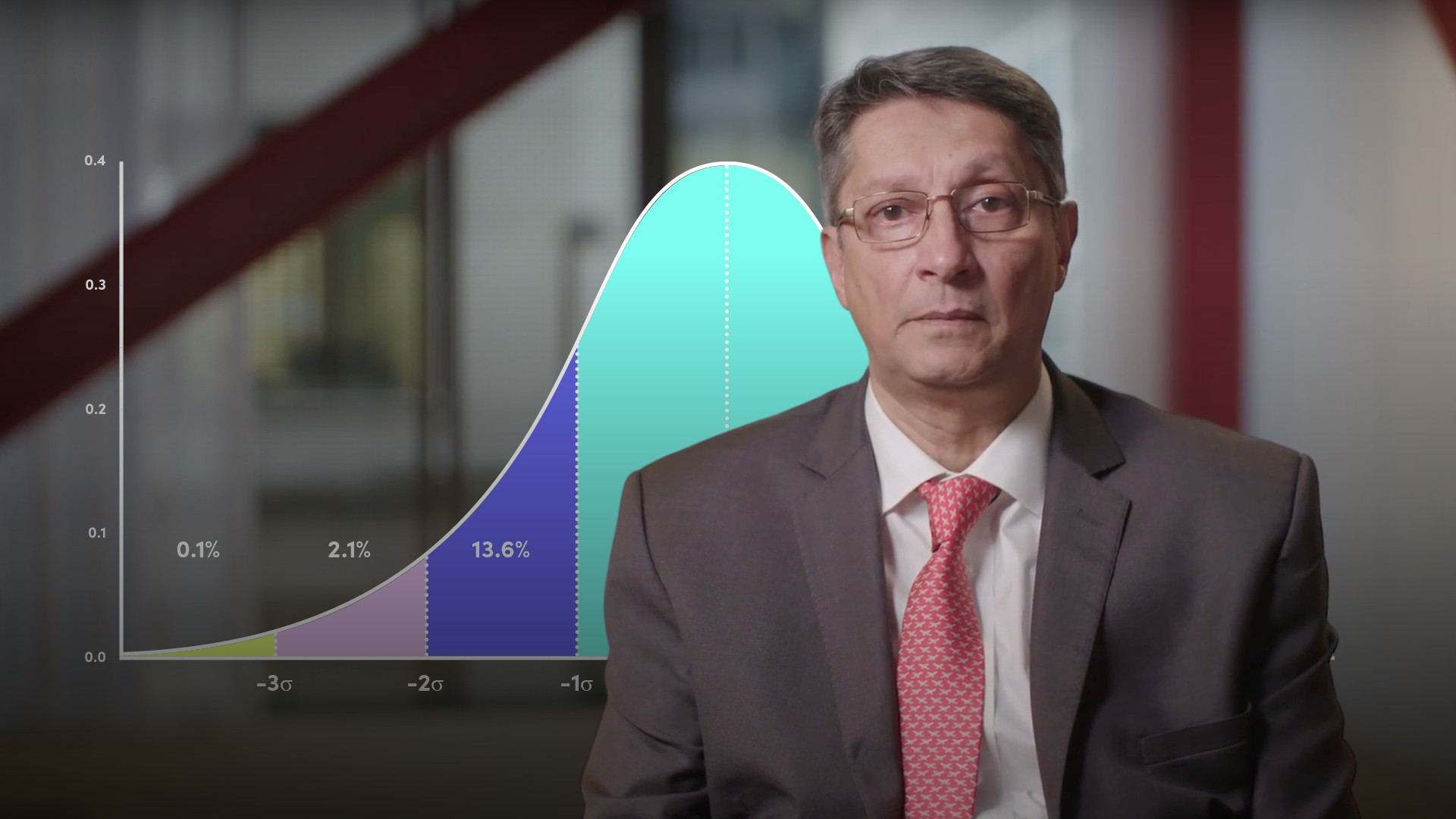05:42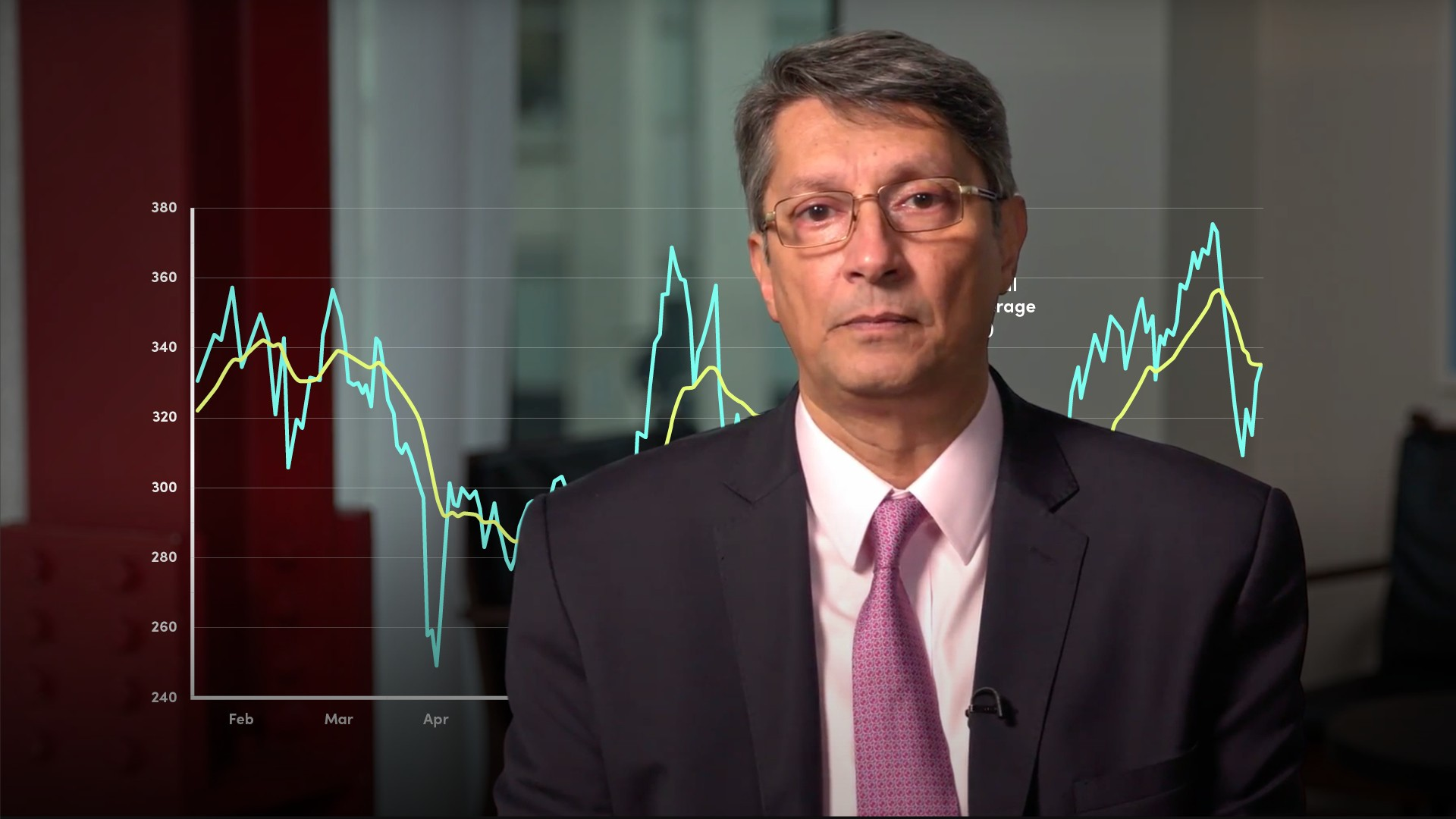05:11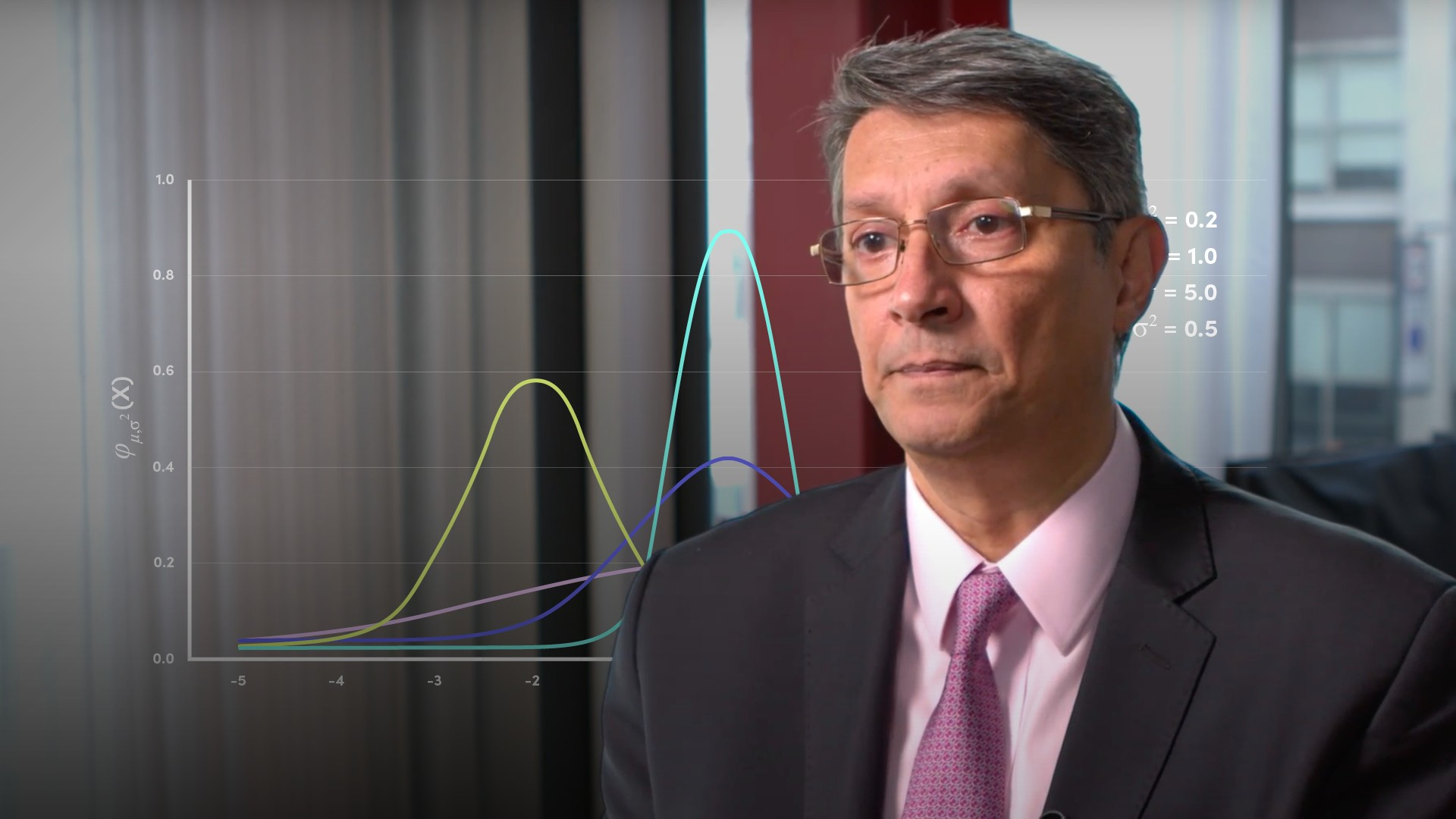04:49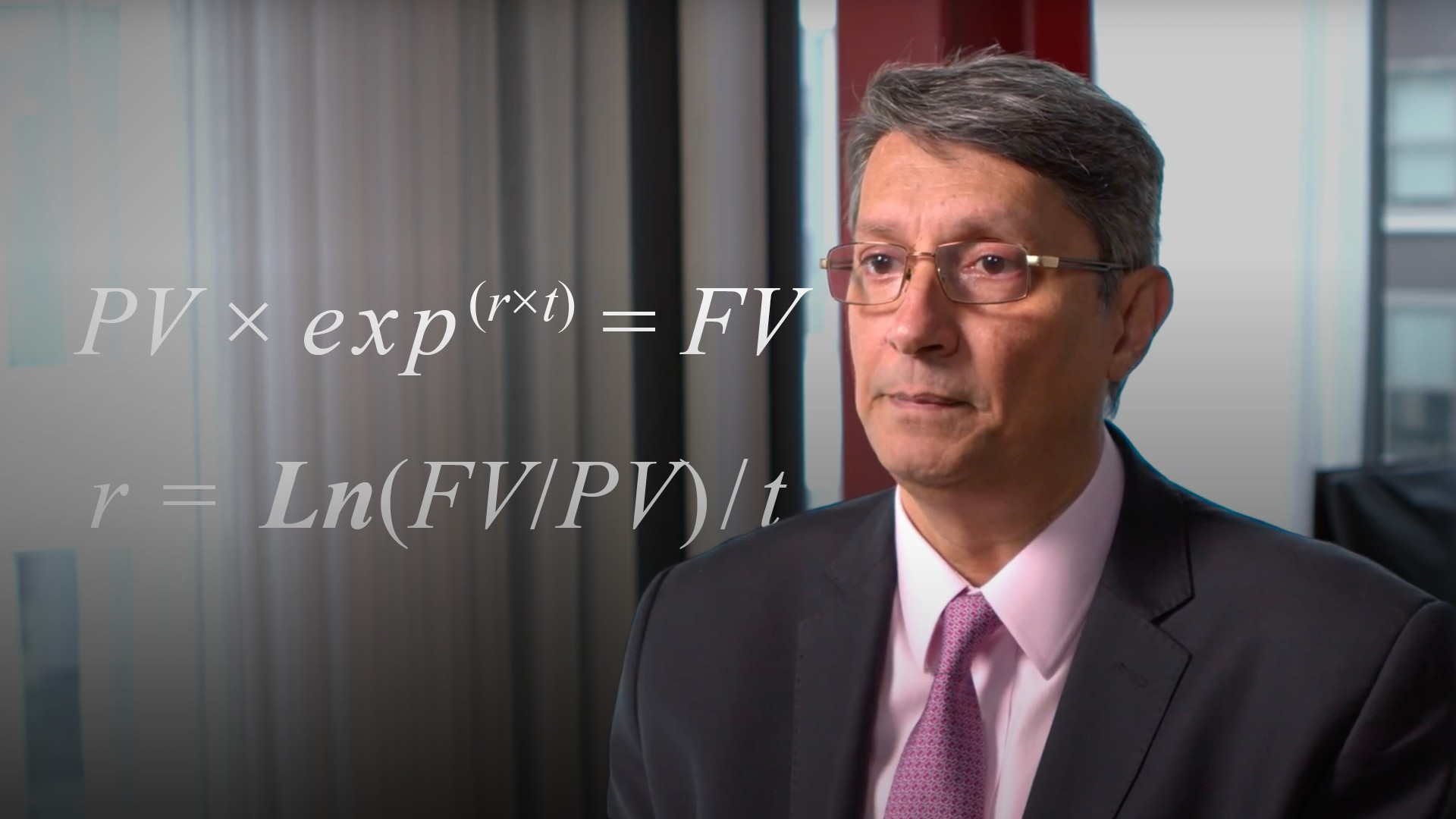03:58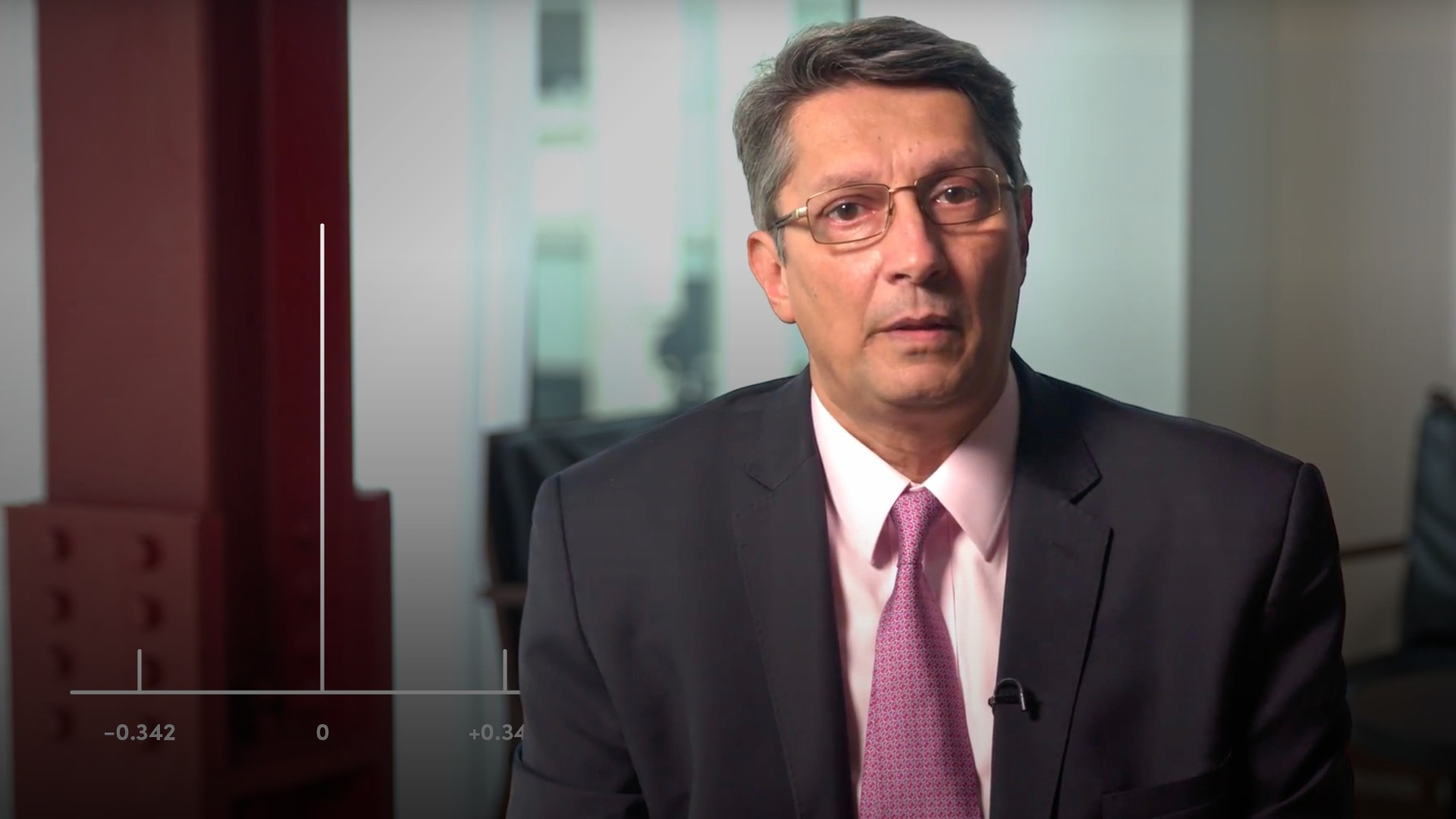06:44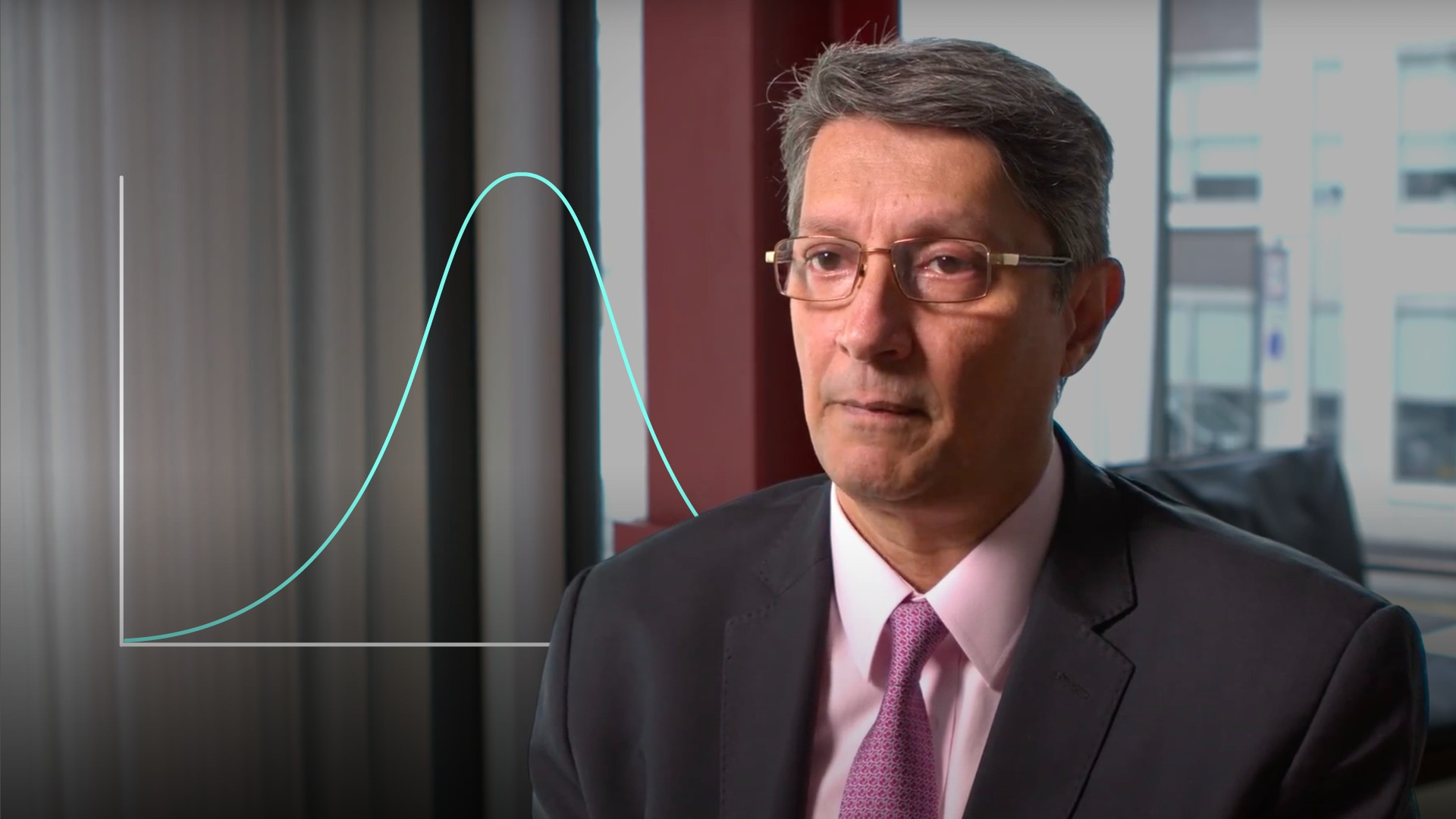03:49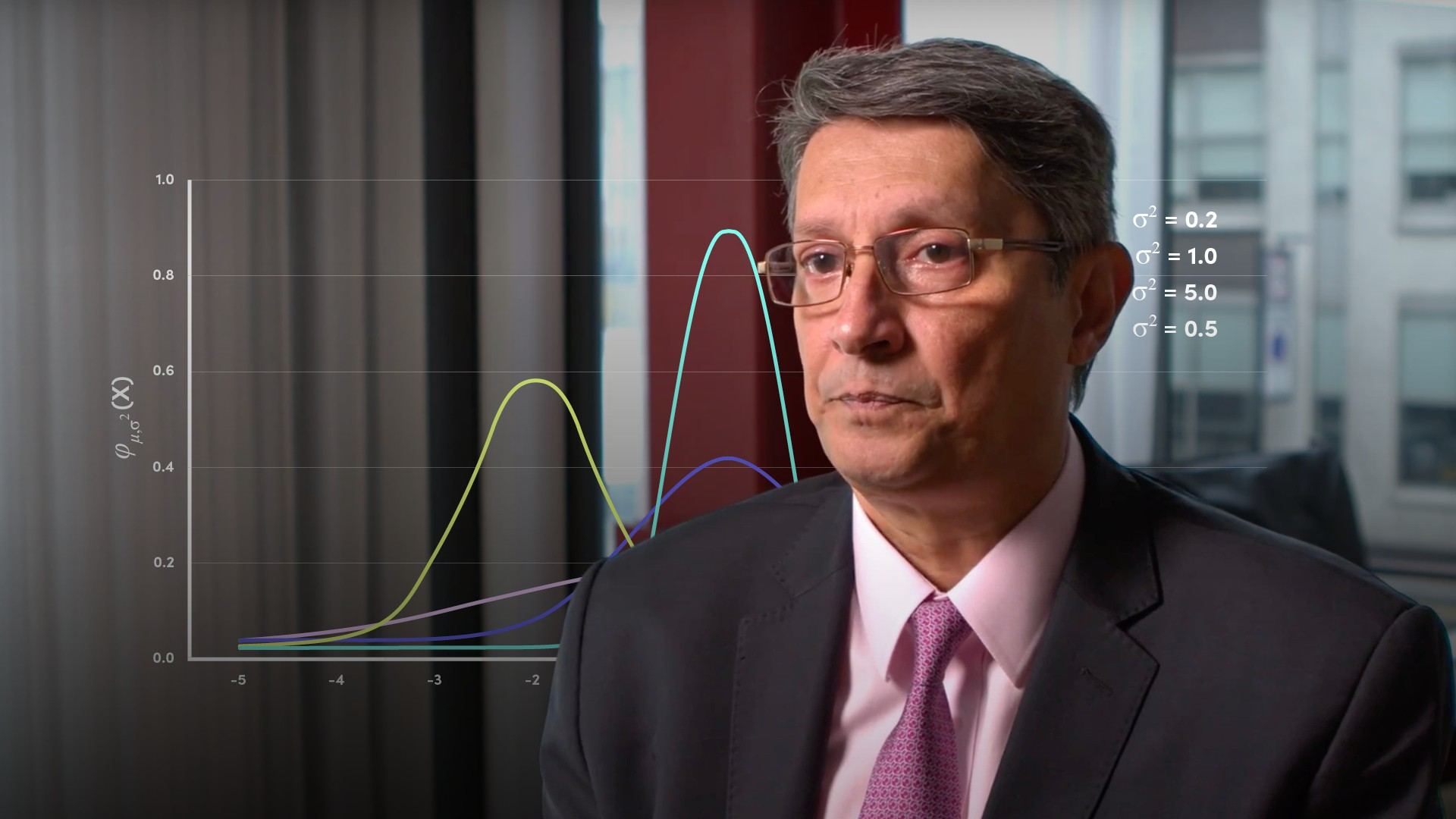04:57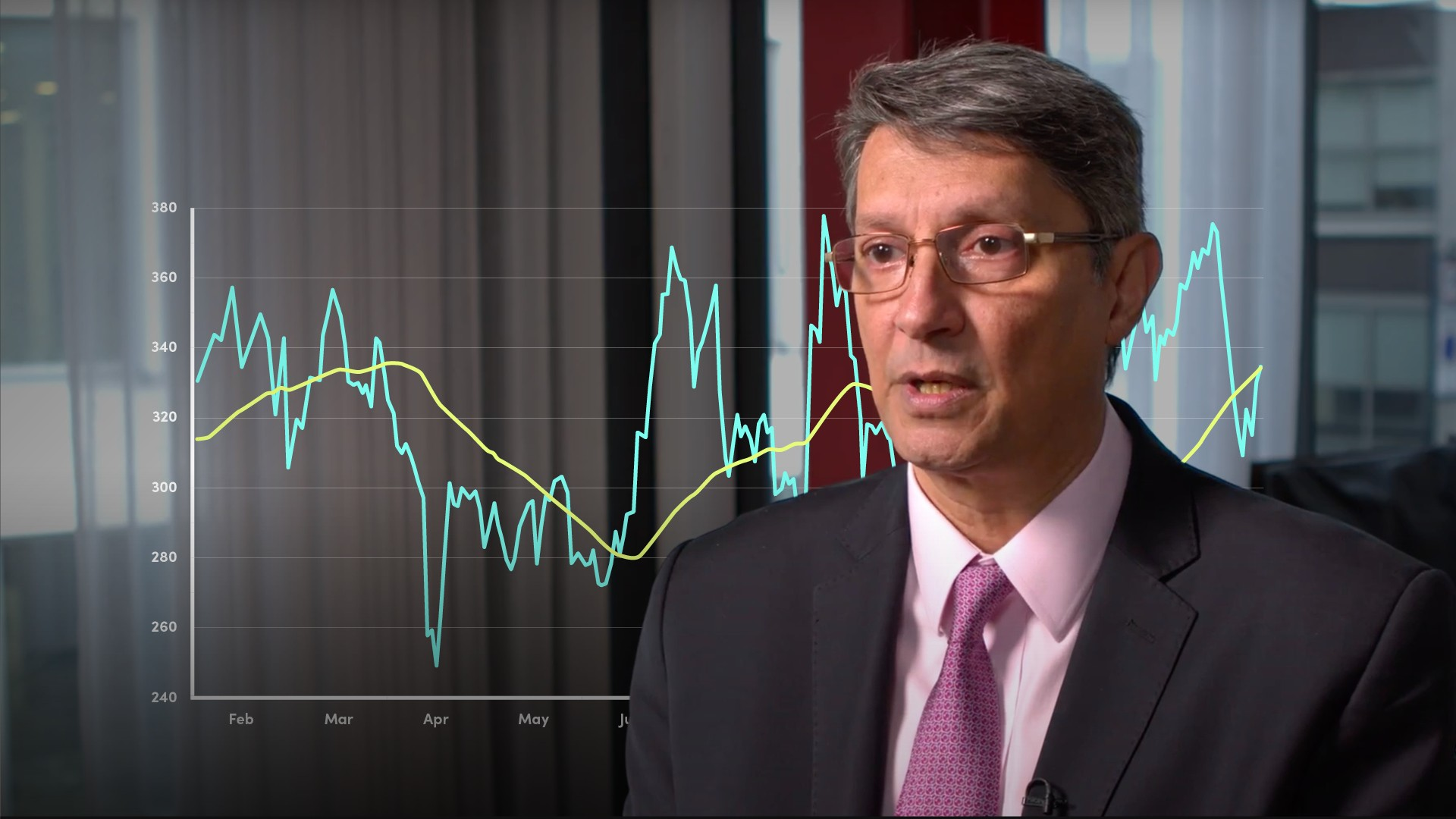04:00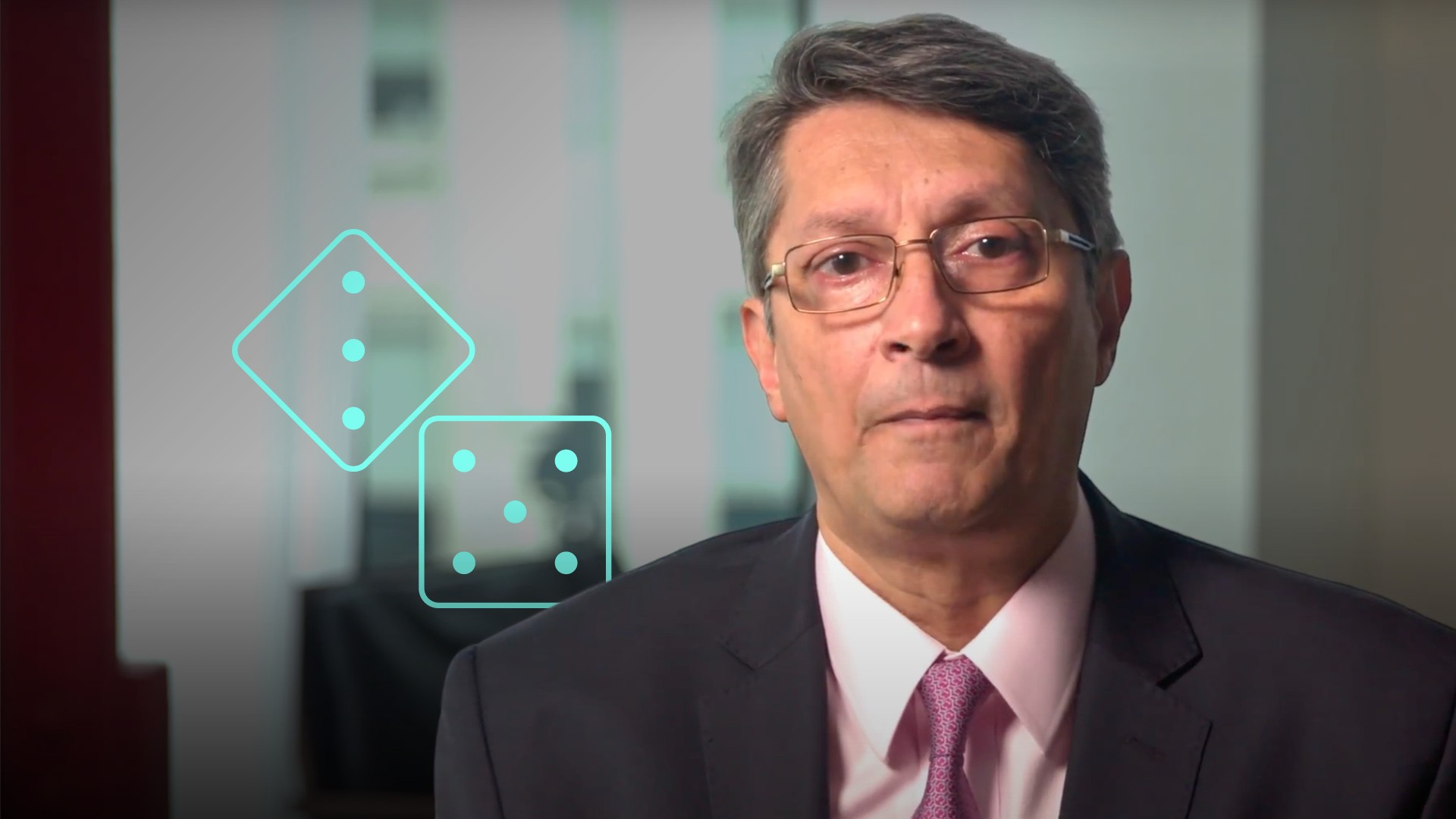04:11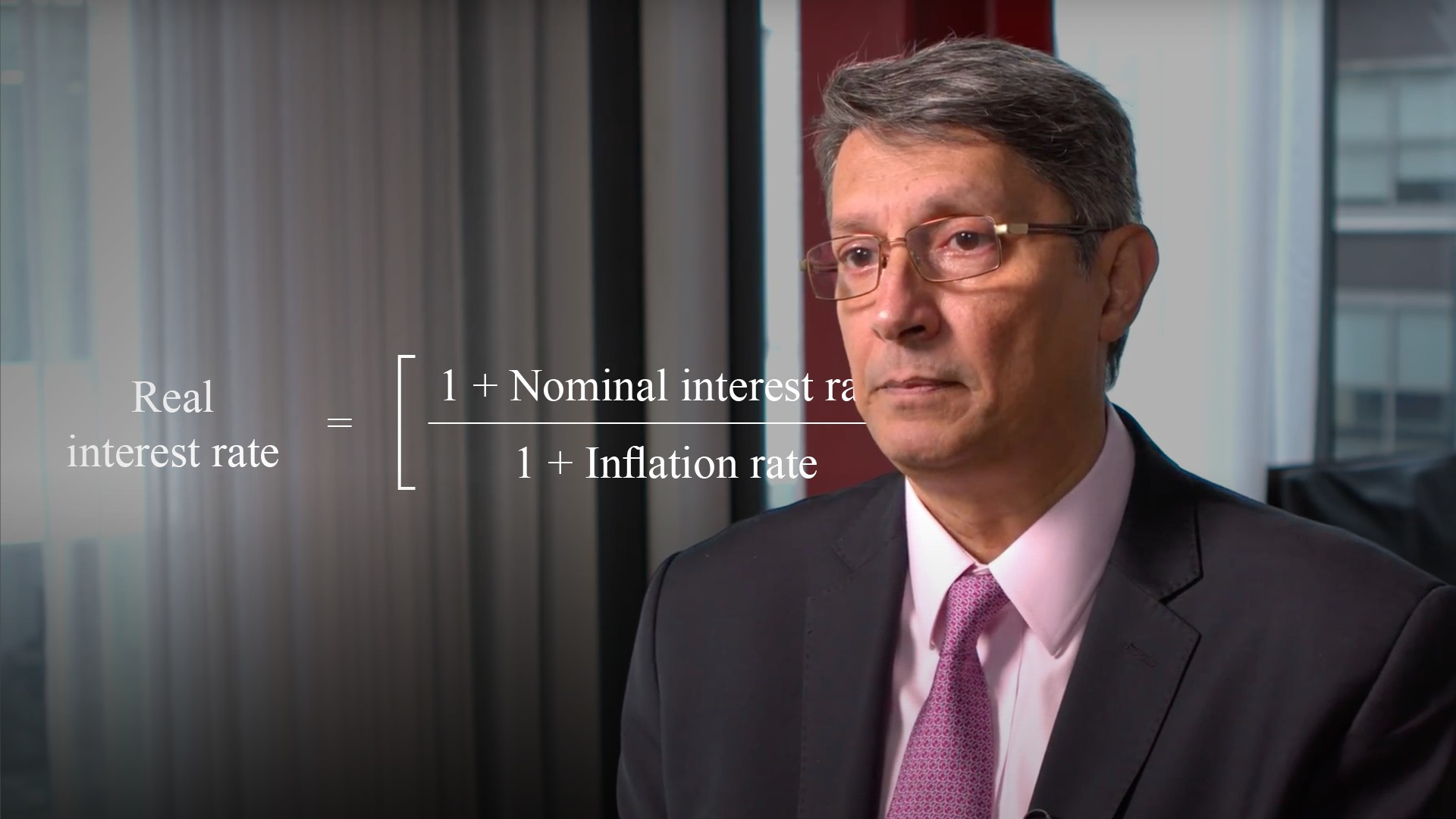04:42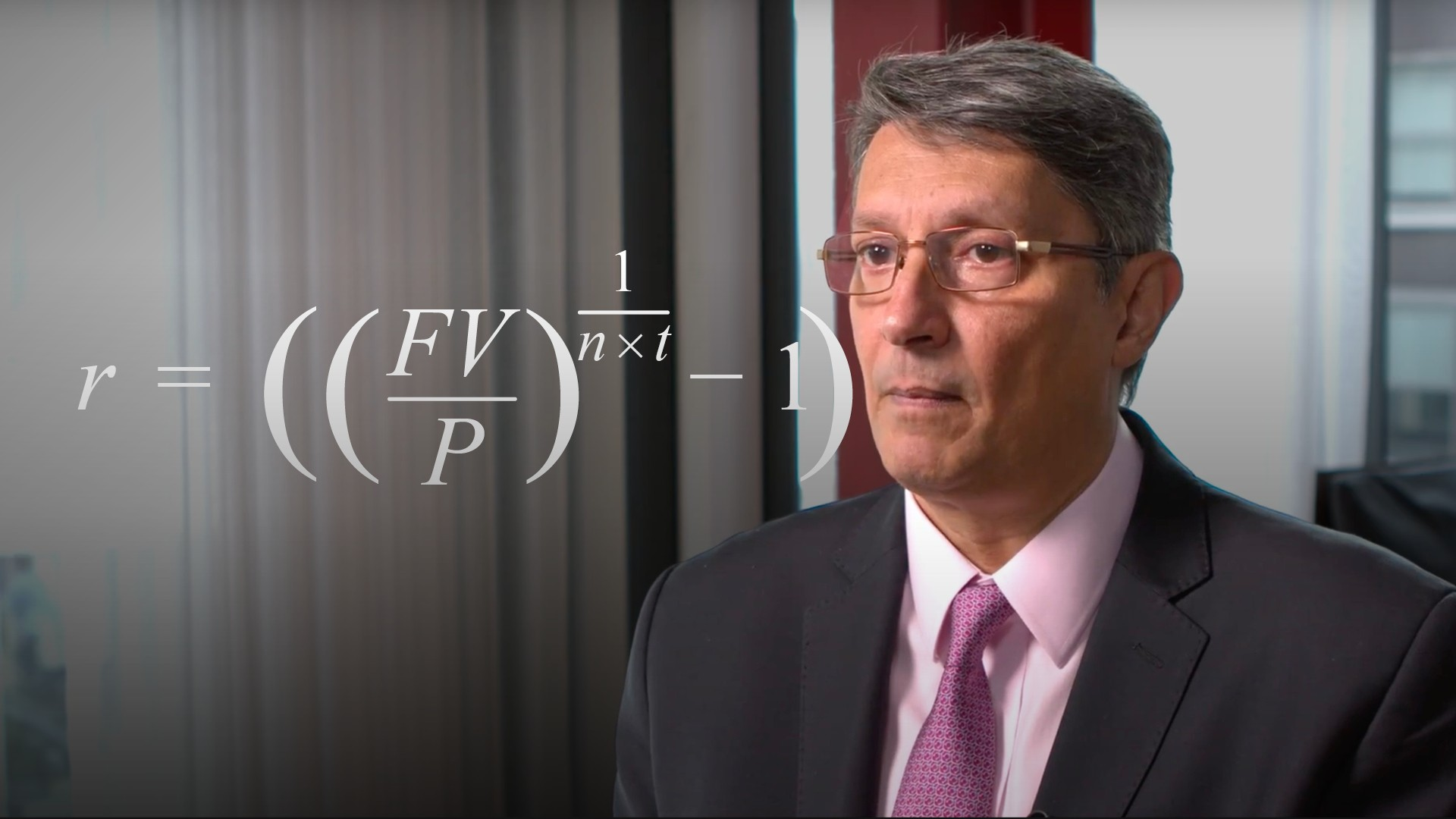05:03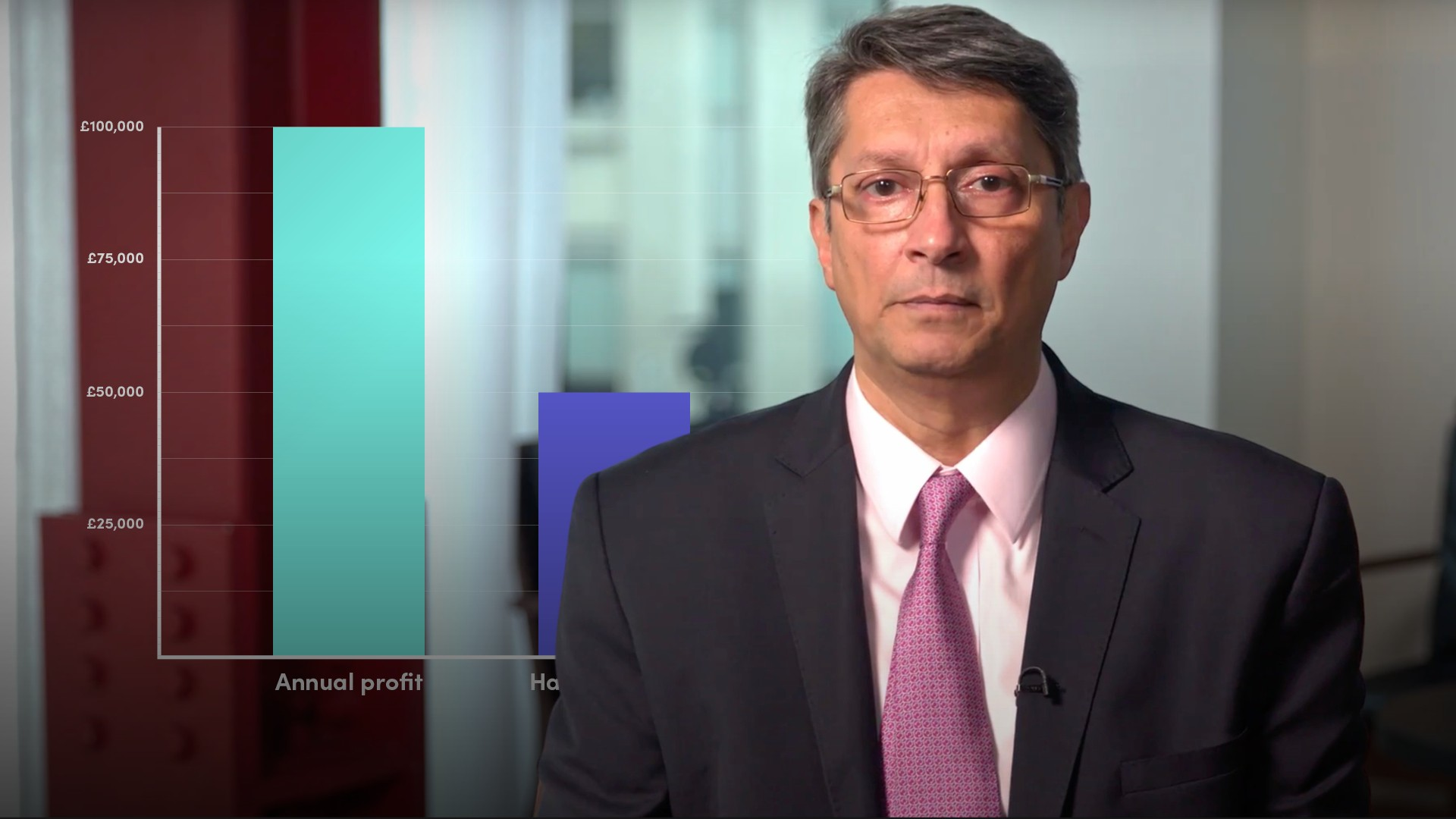04:37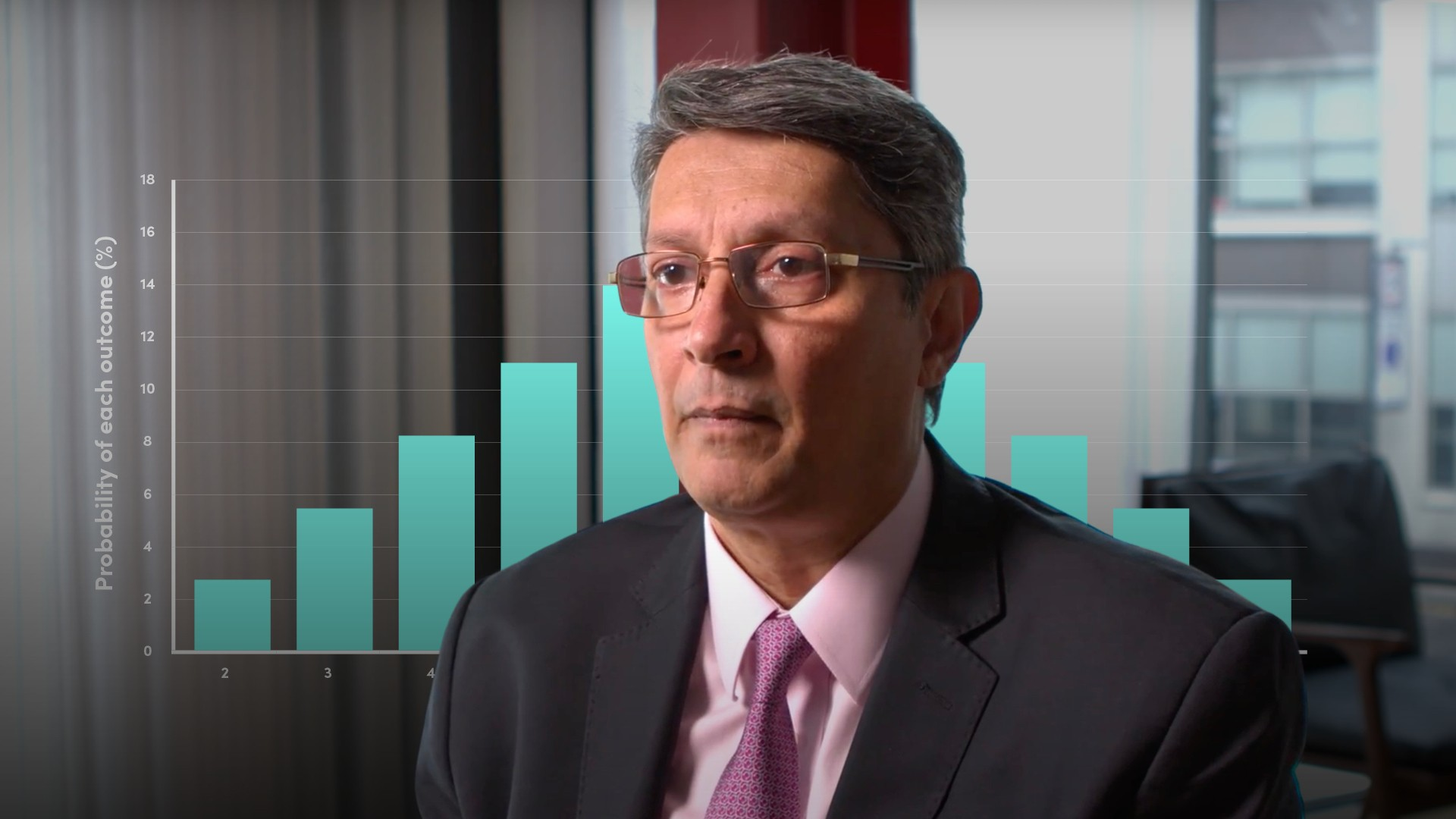04:36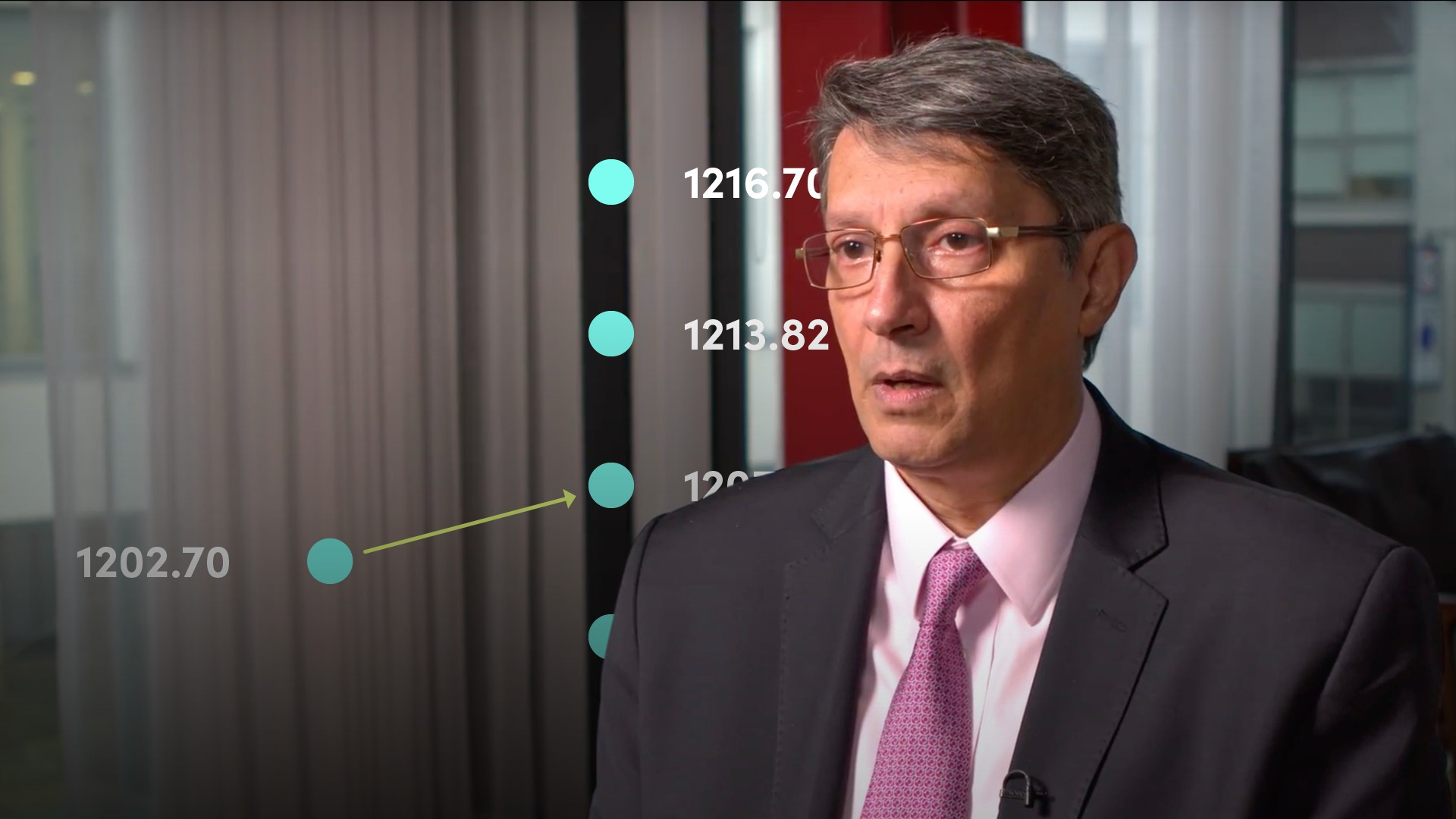06:35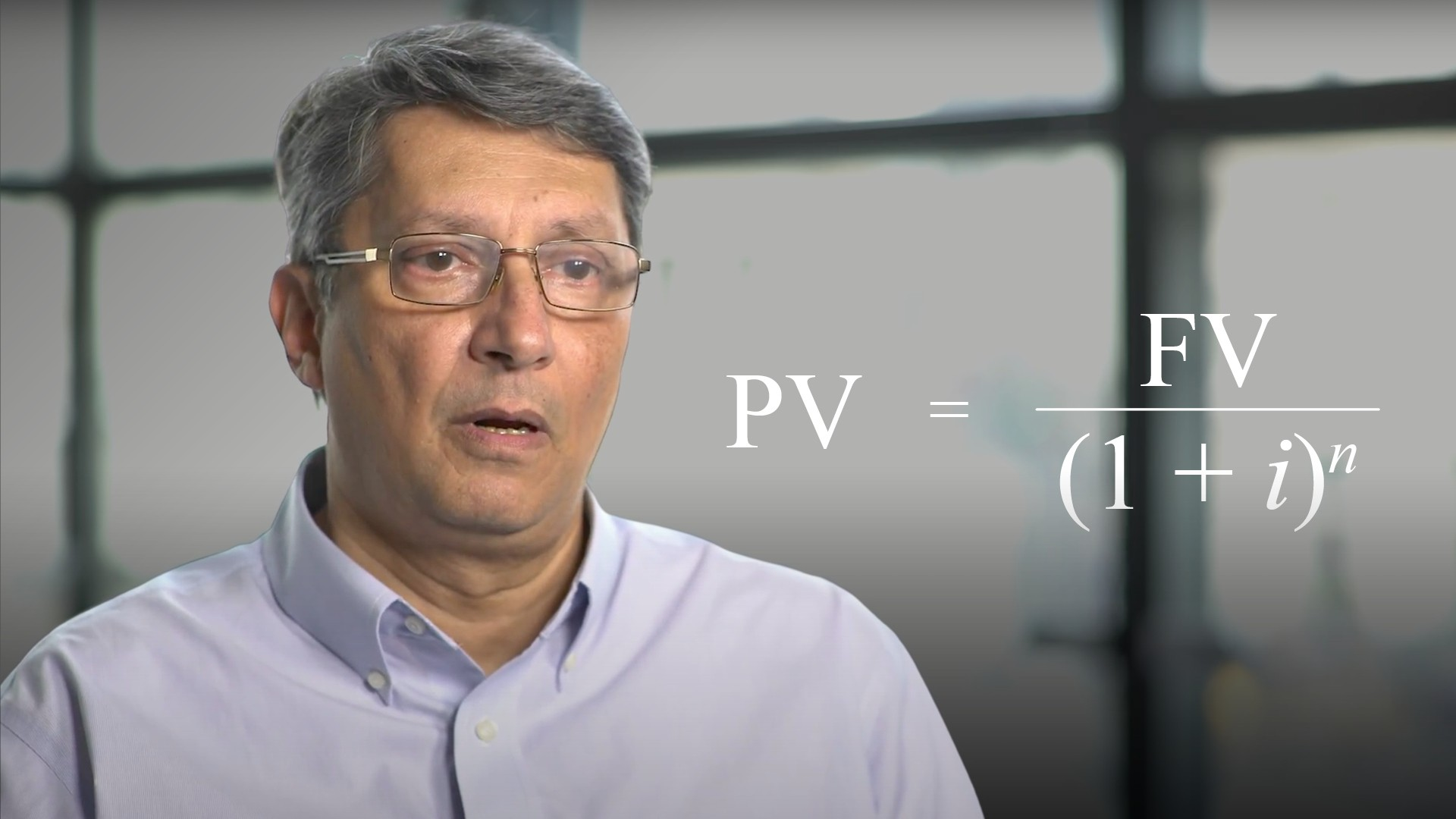05:00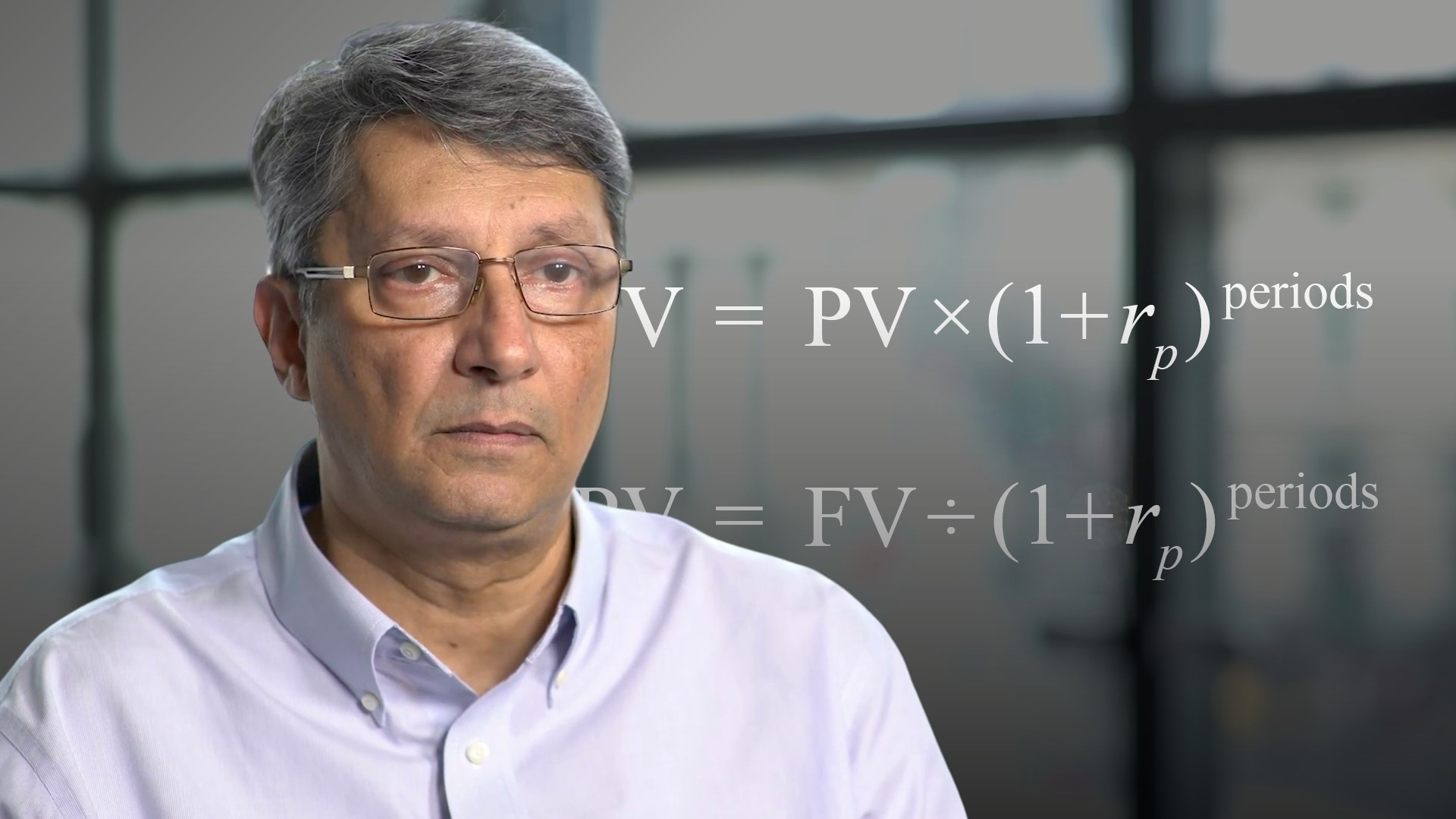03:10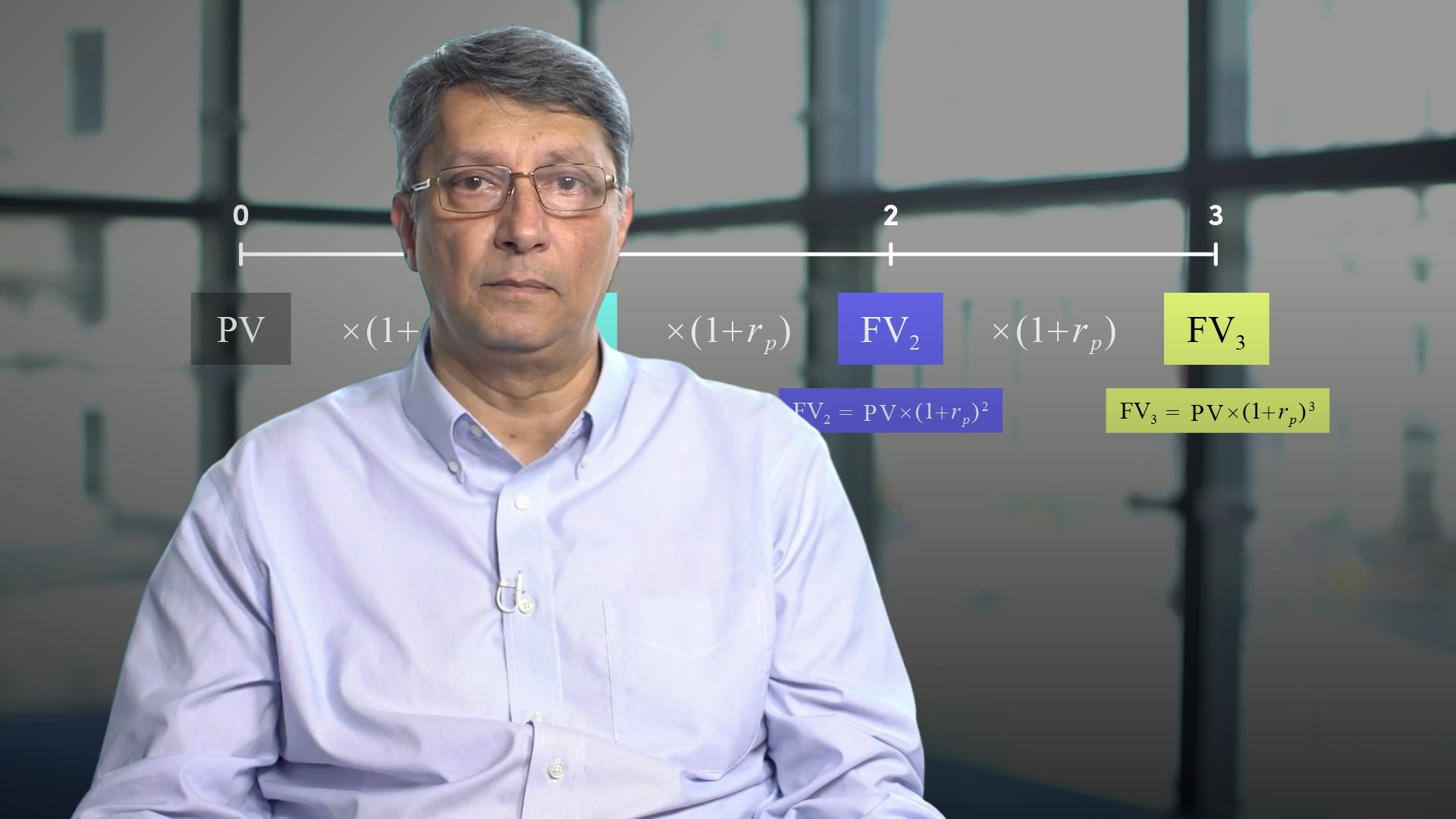06:08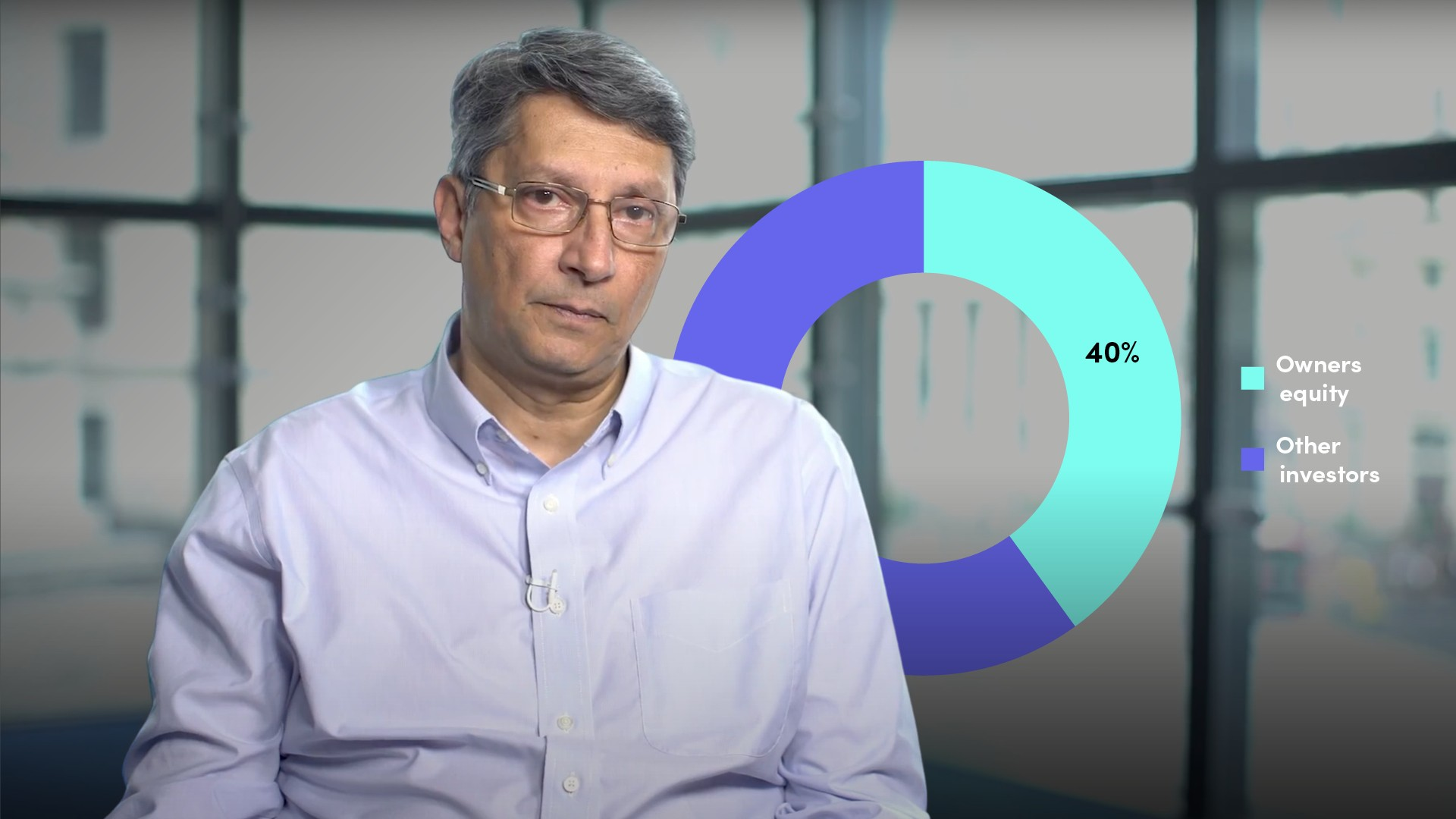05:23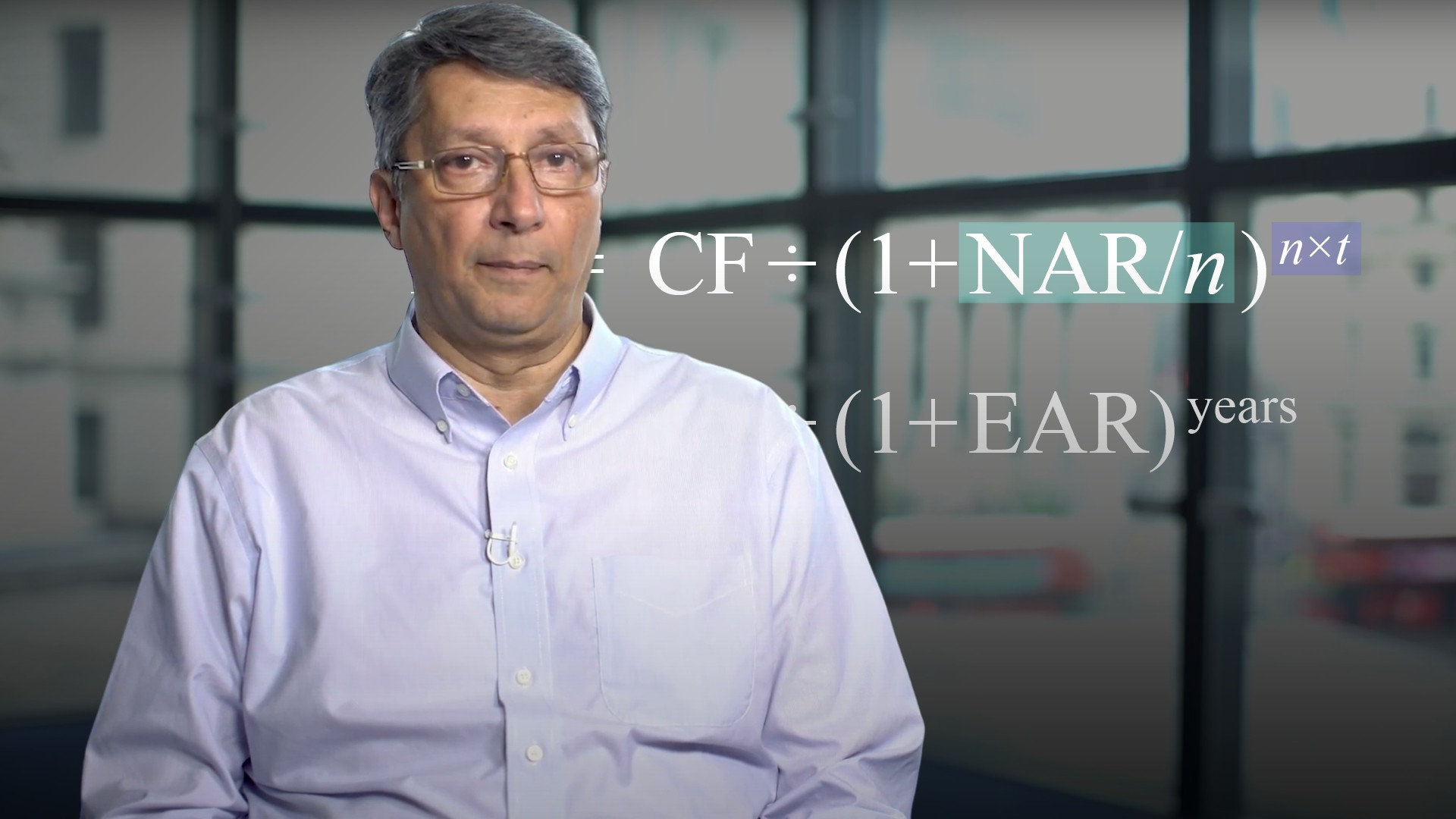04:04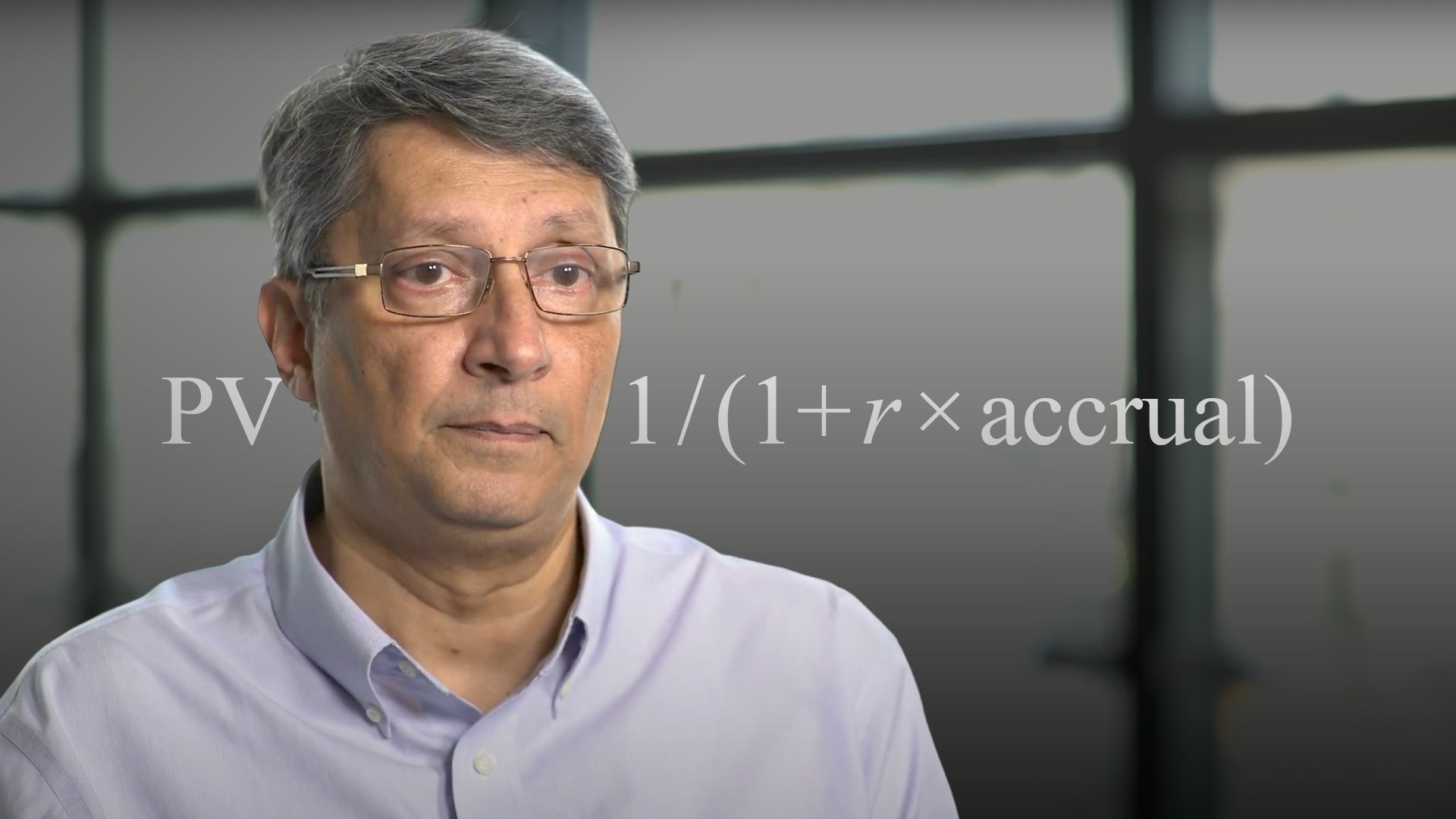03:46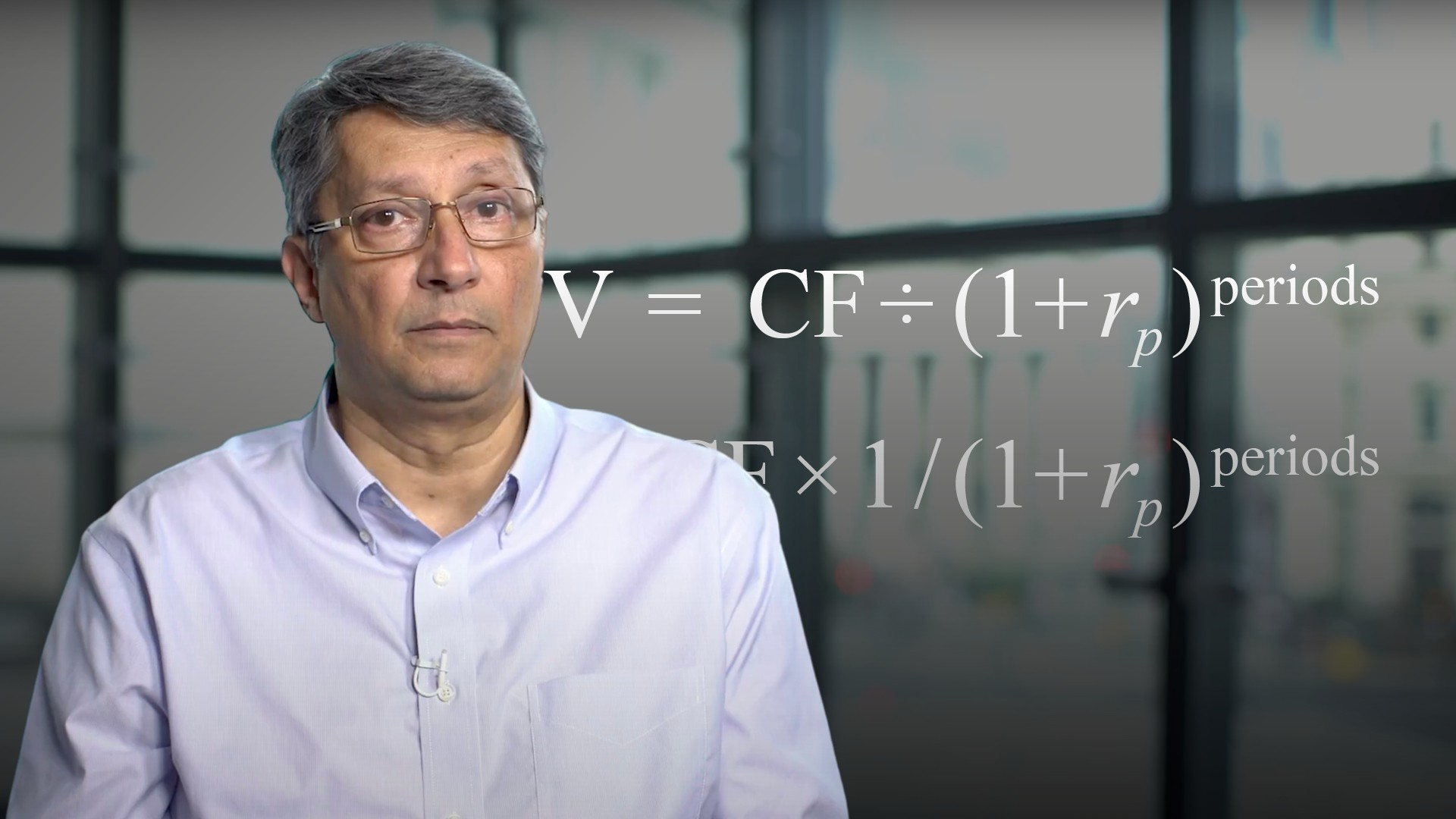04:19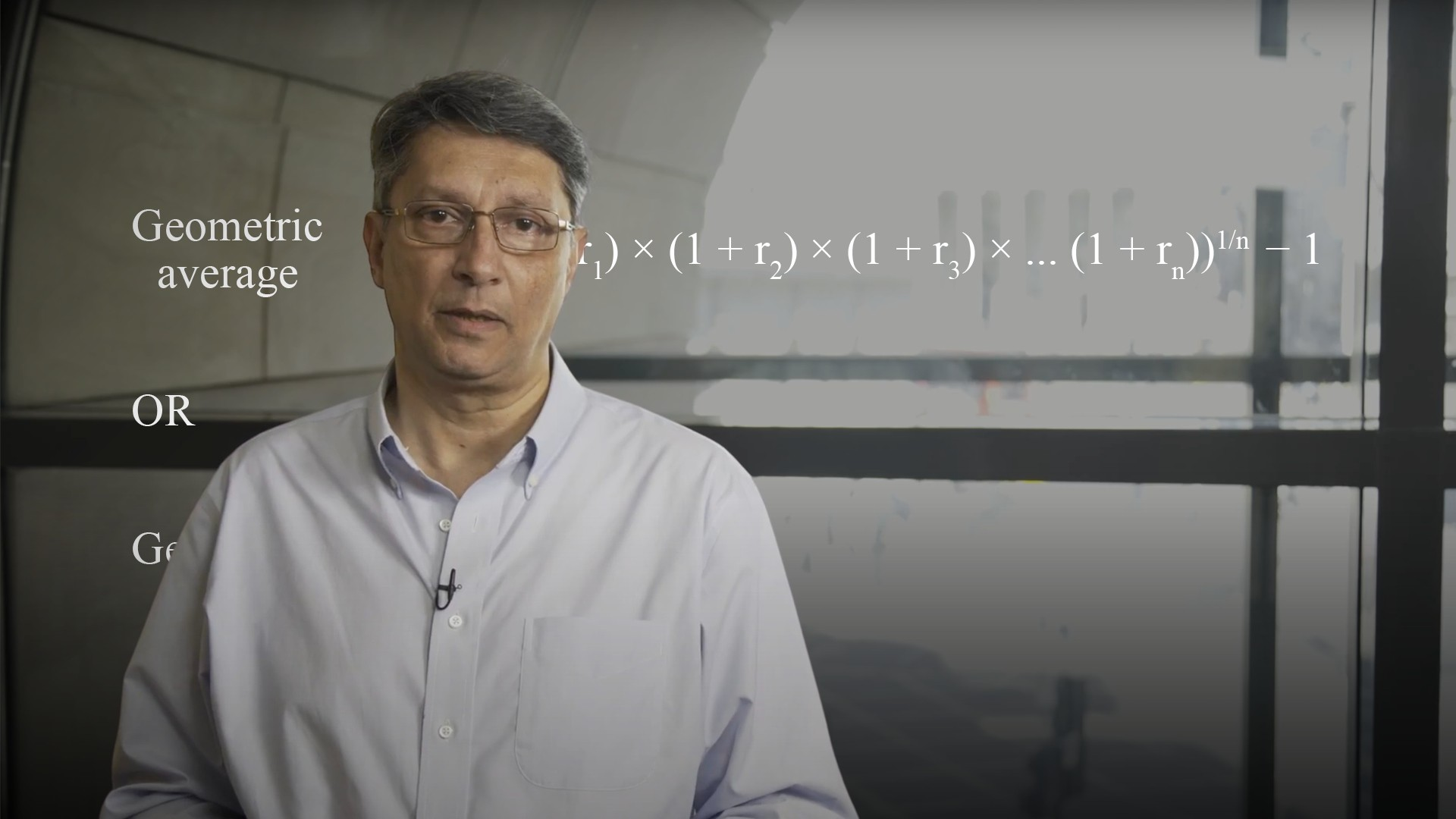04:41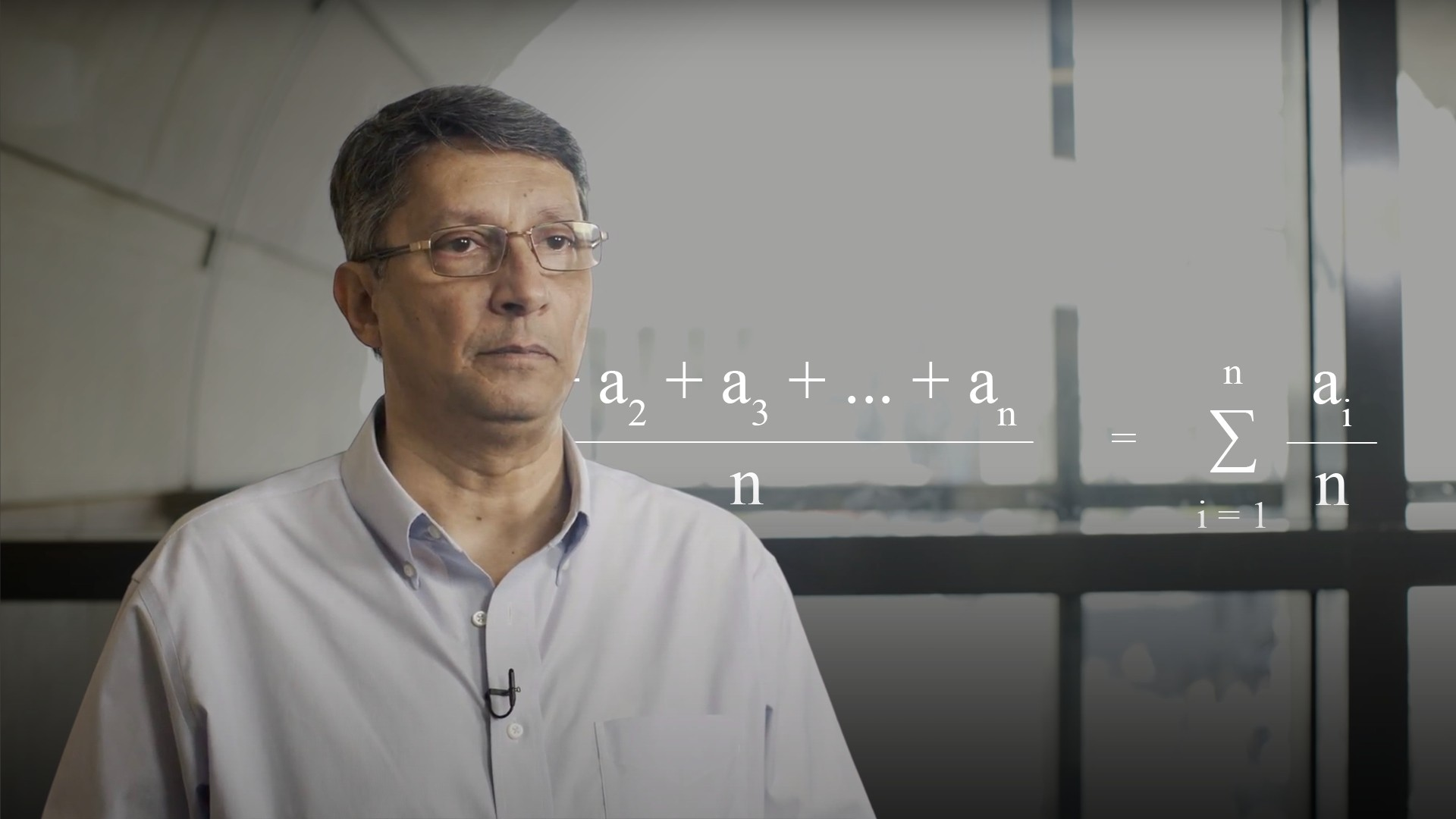04:16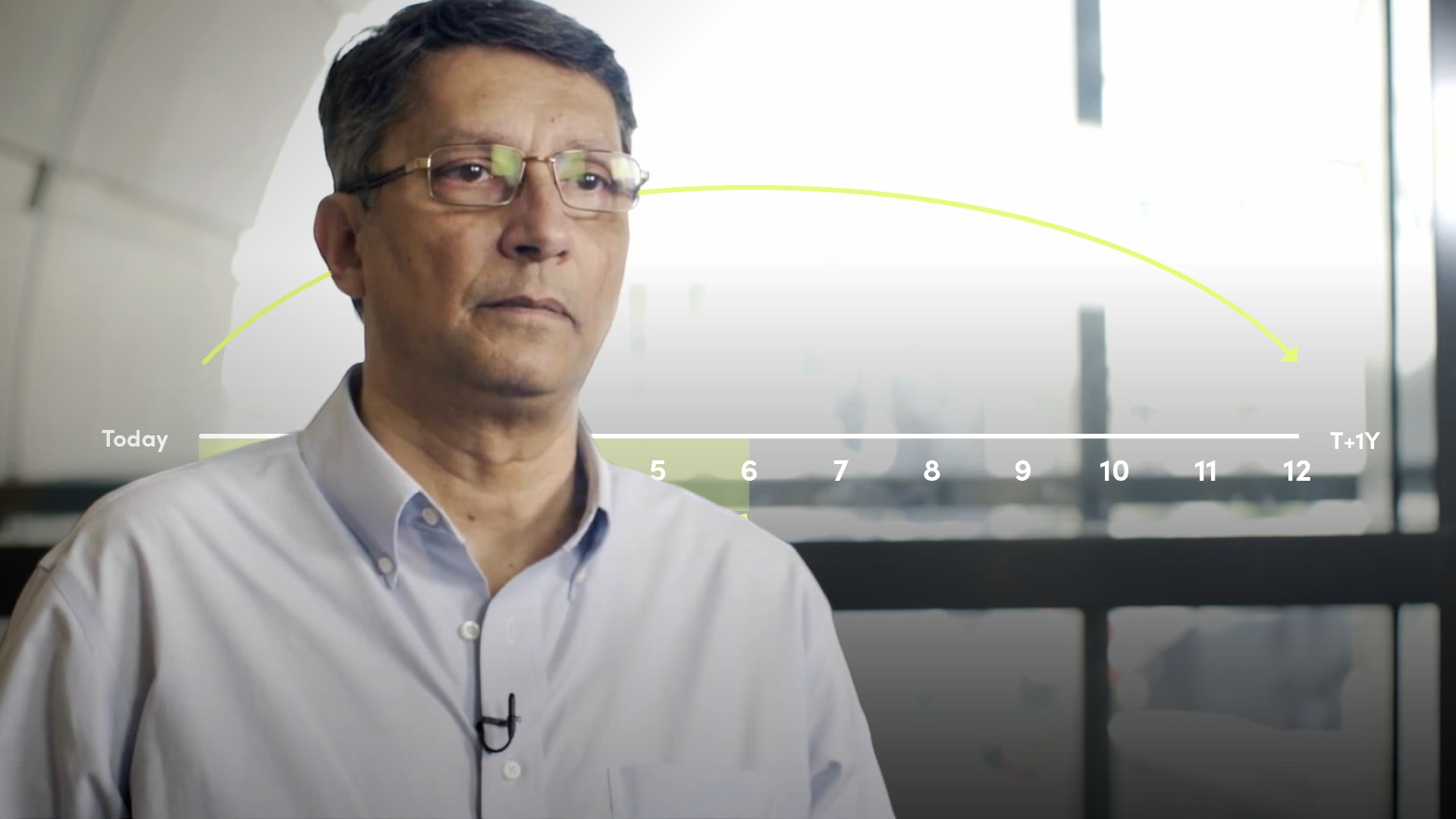05:24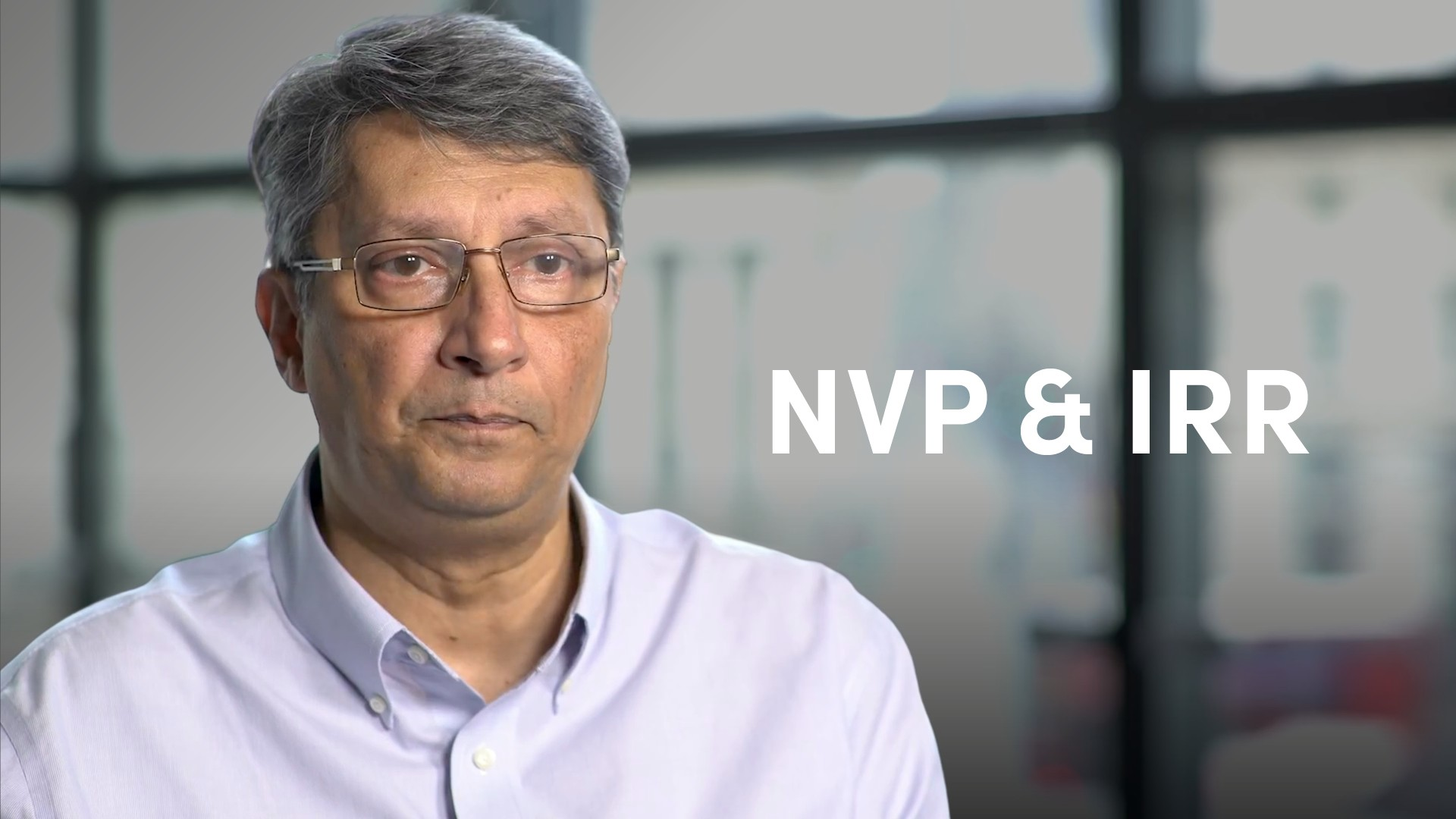05:39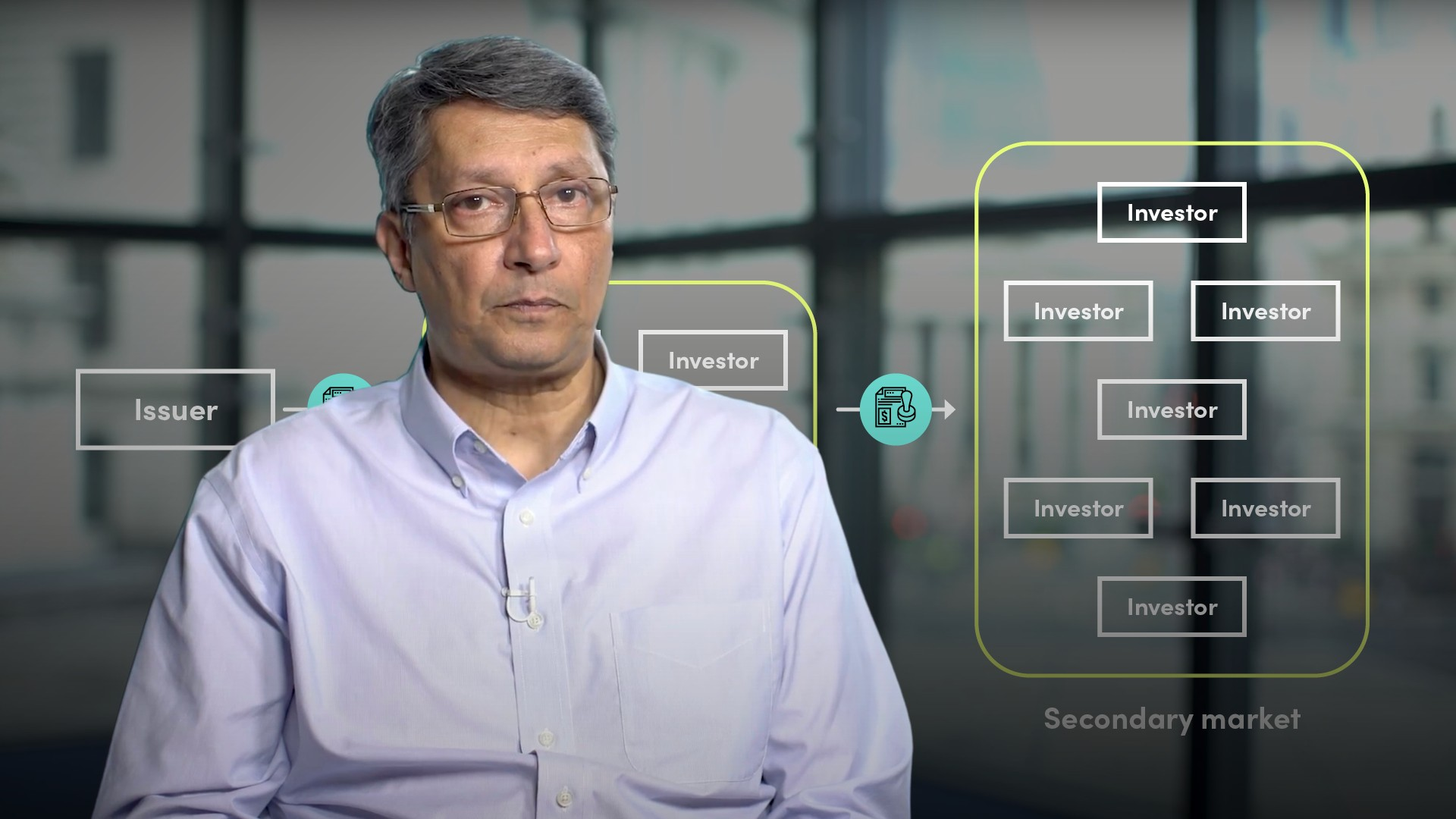06:13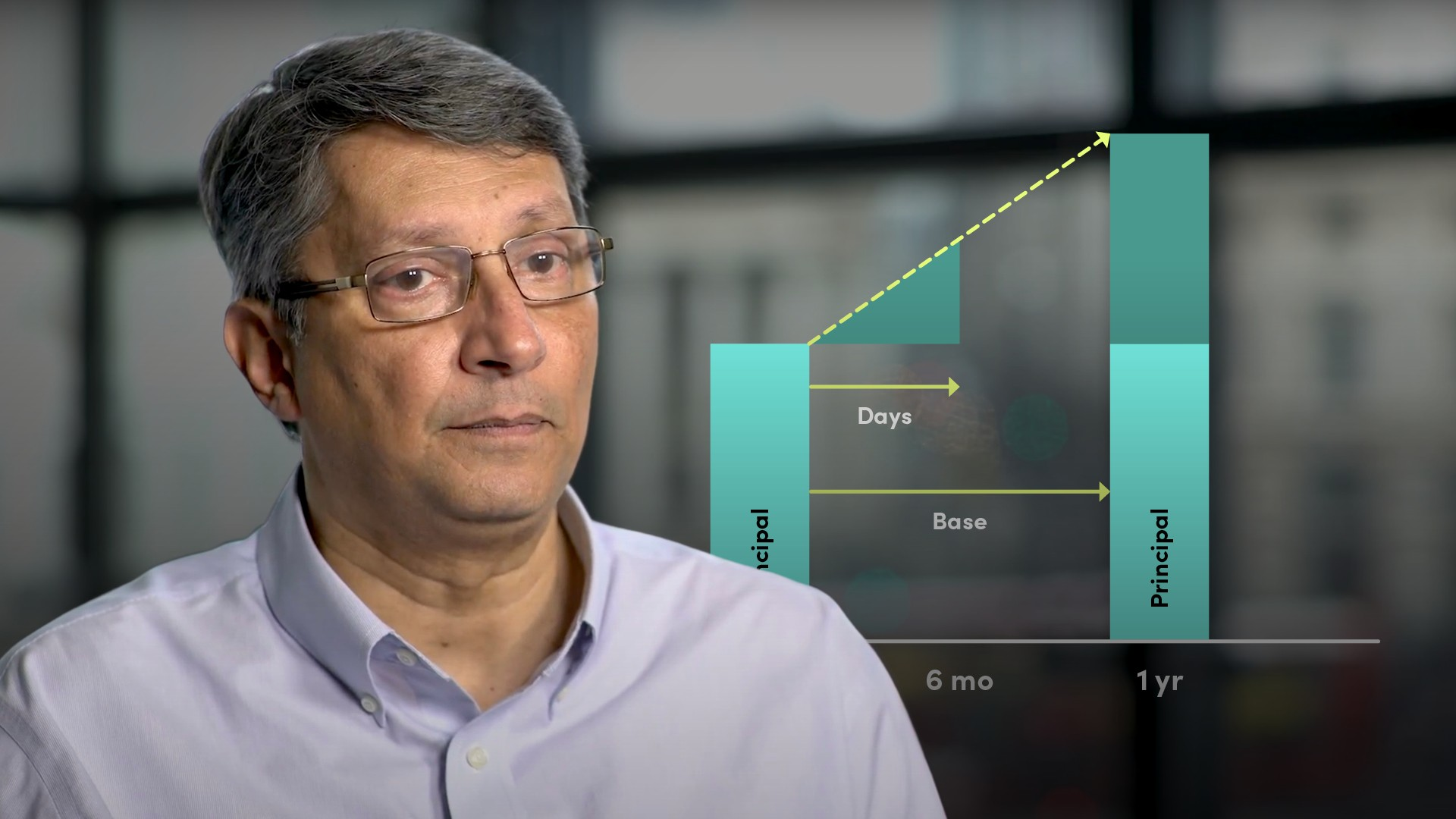05:27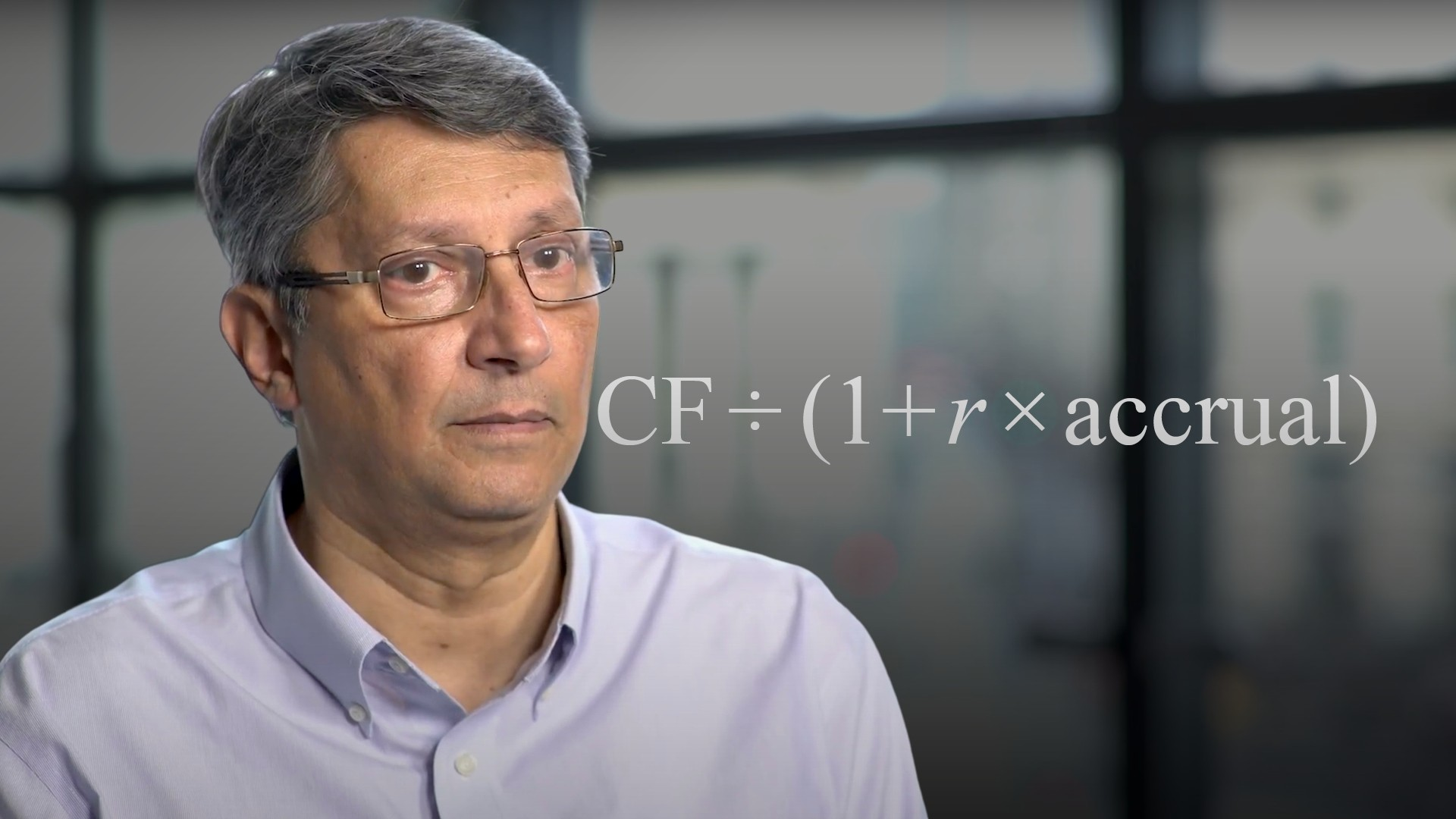03:55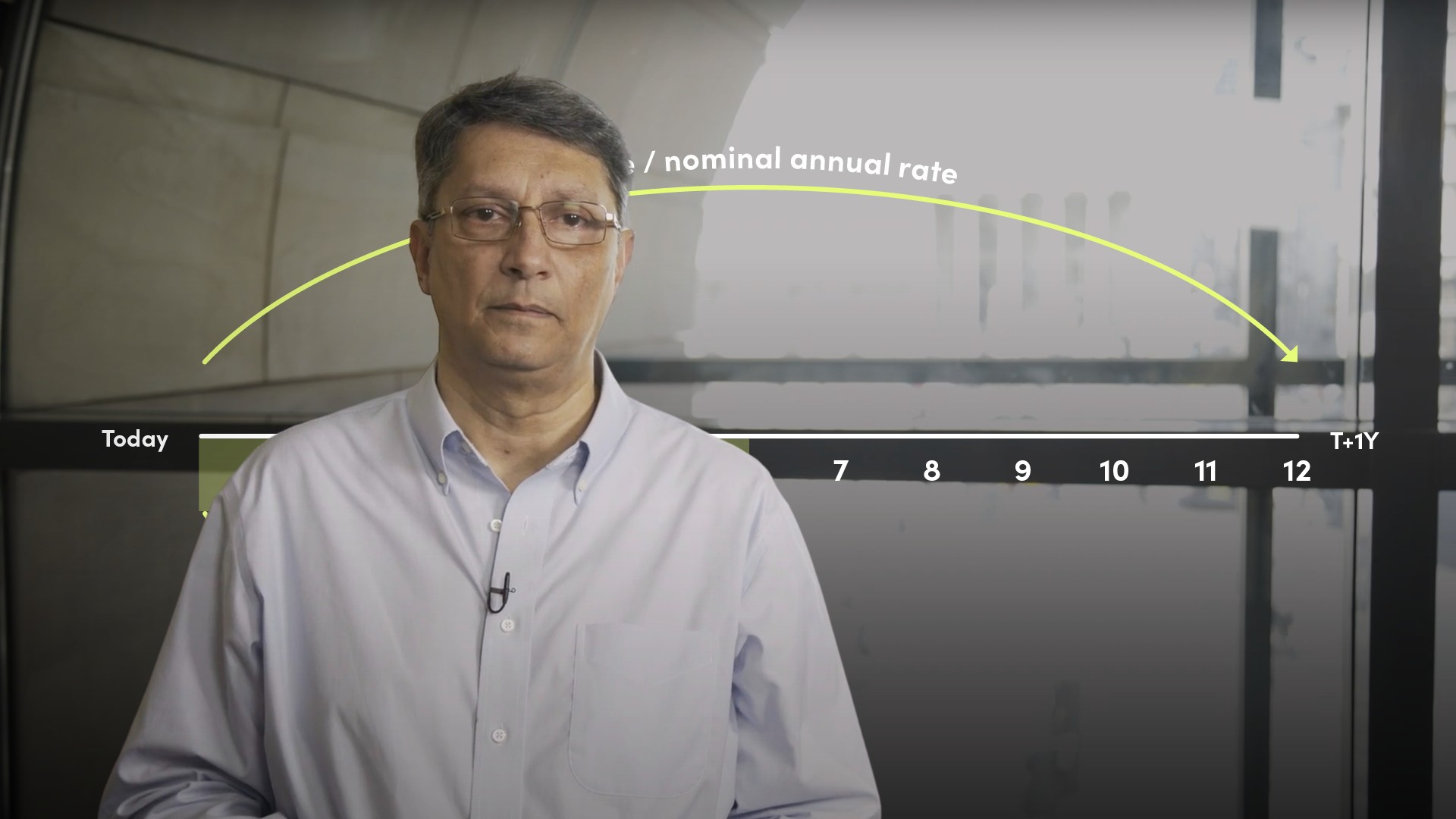07:58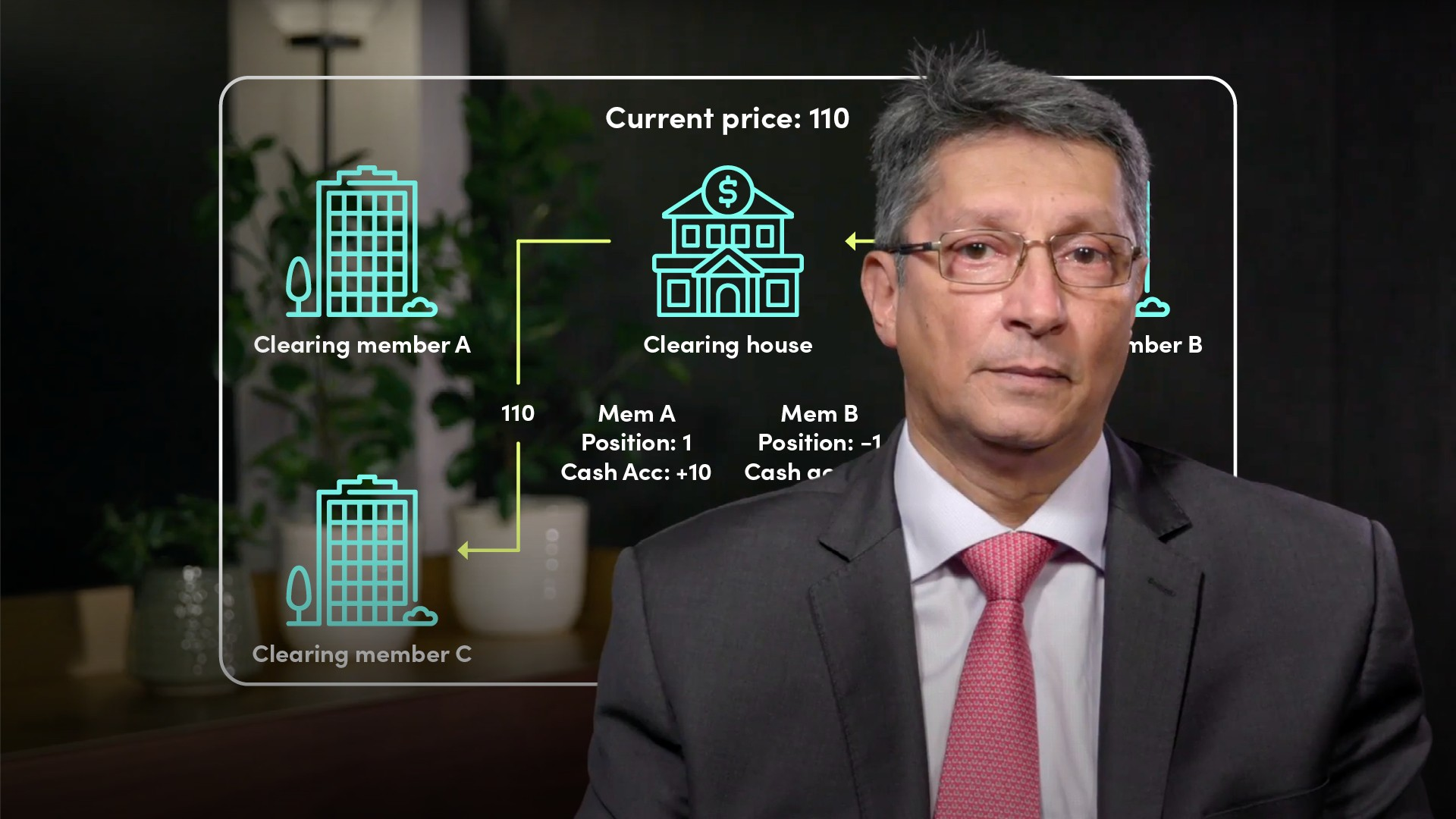05:22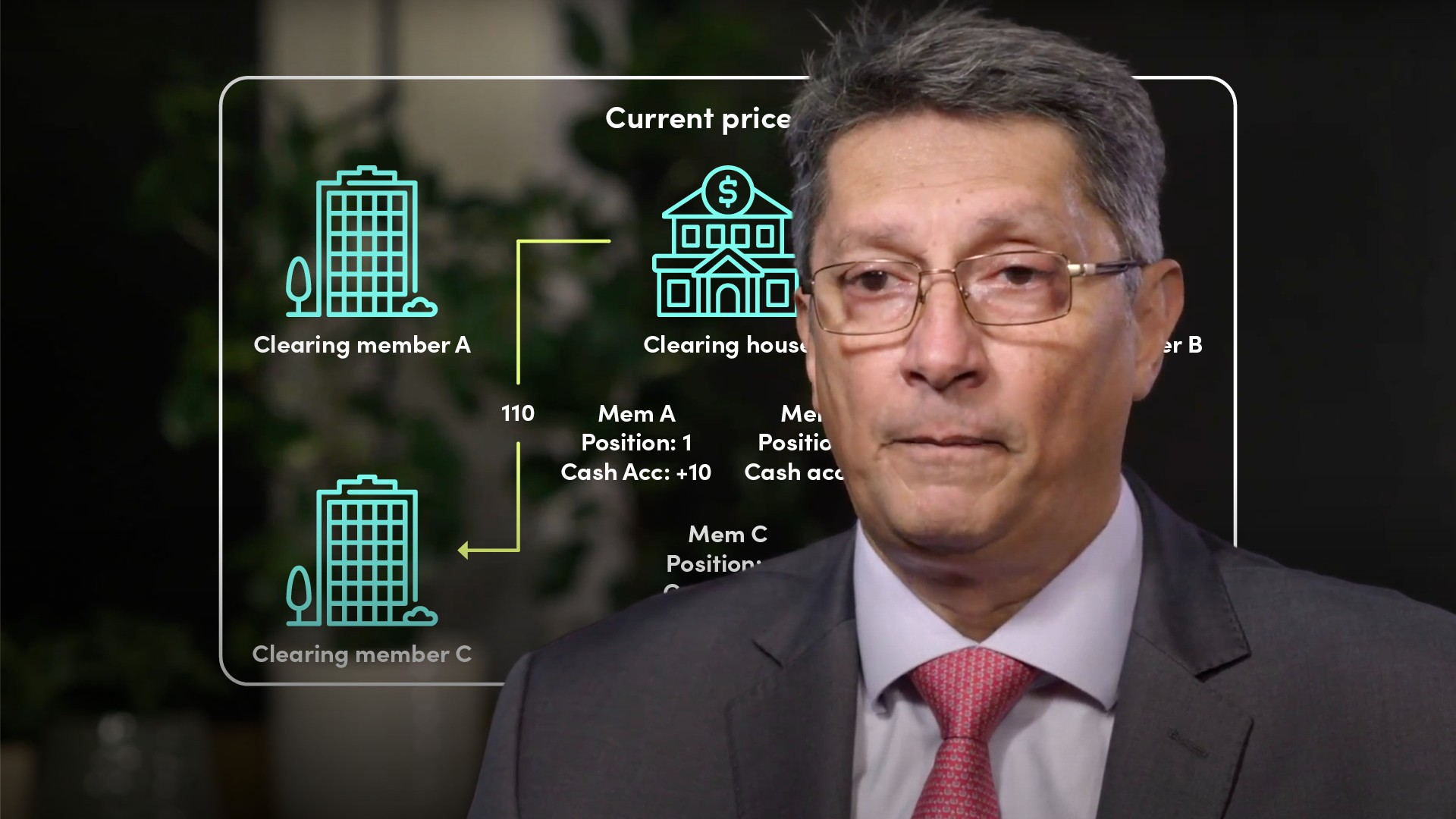05:14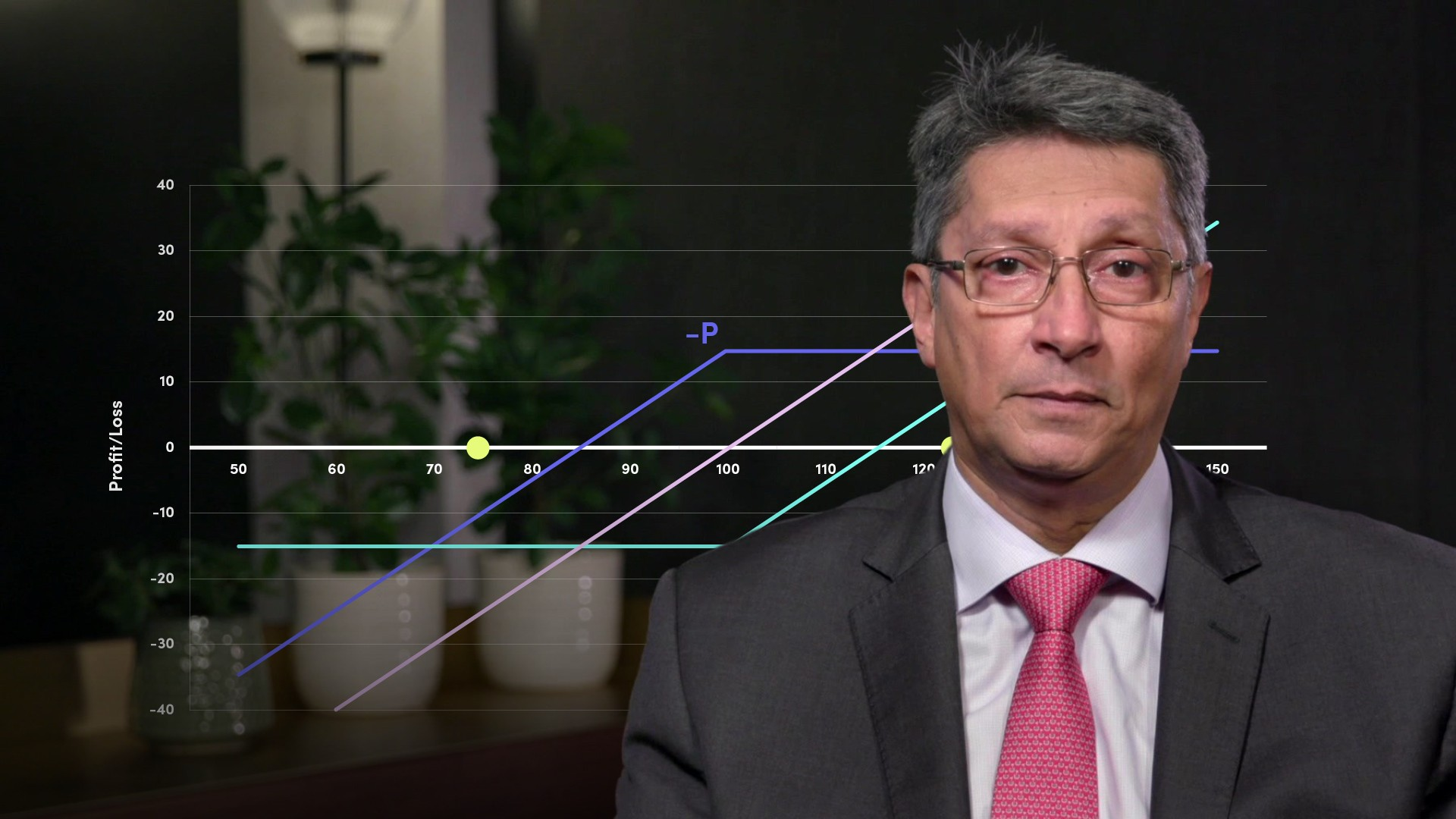06:01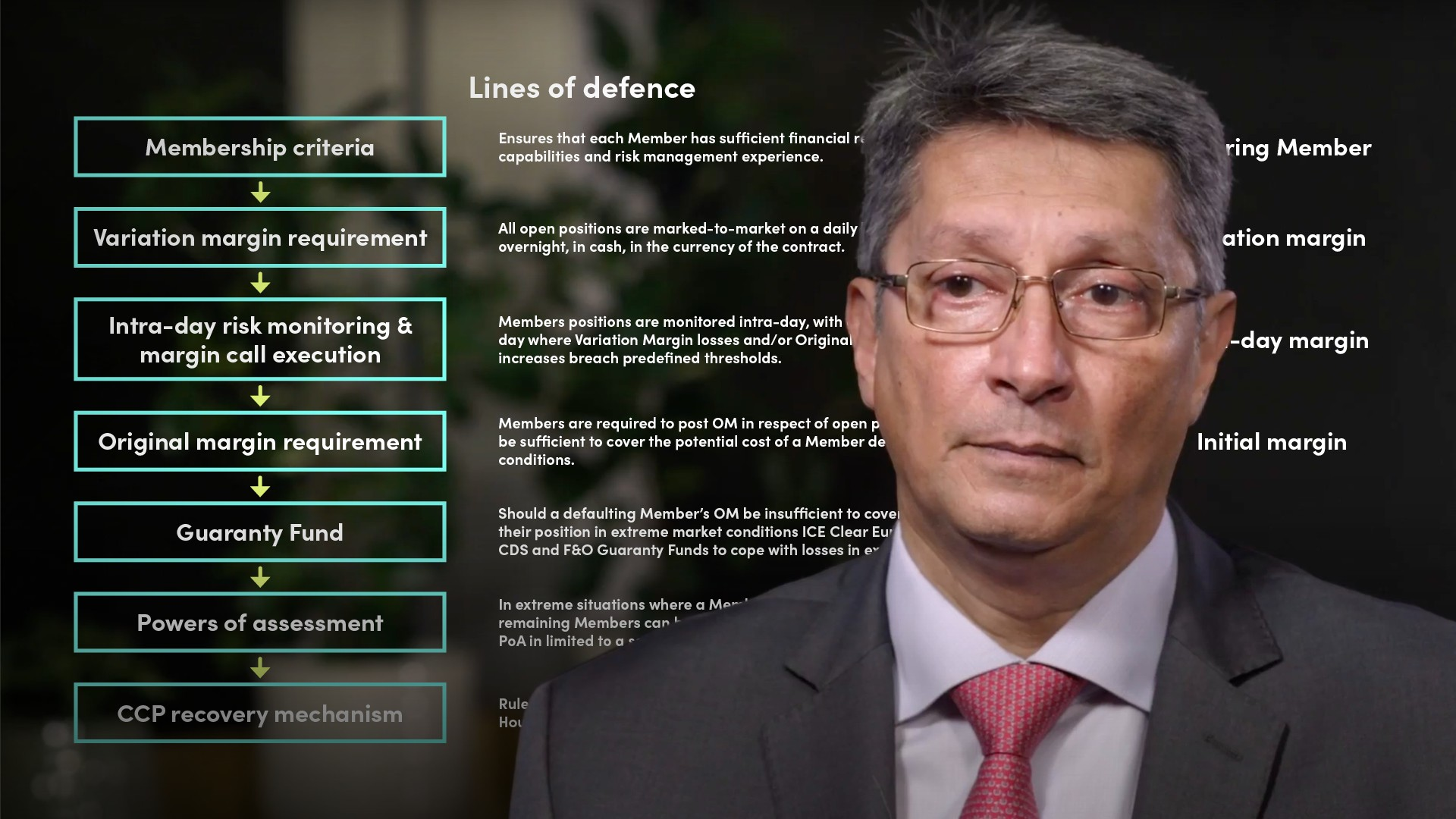06:1306:23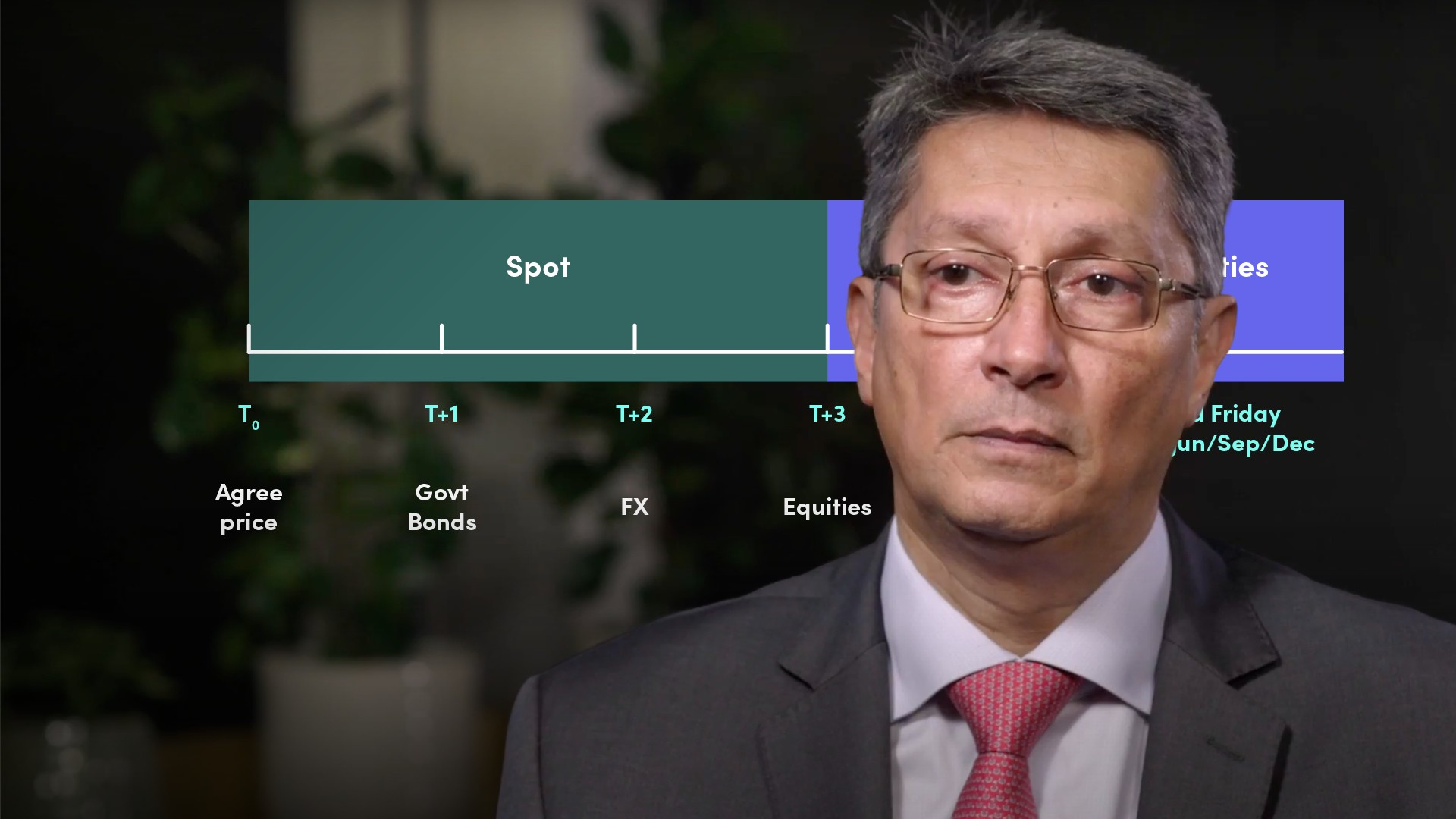05:3807:23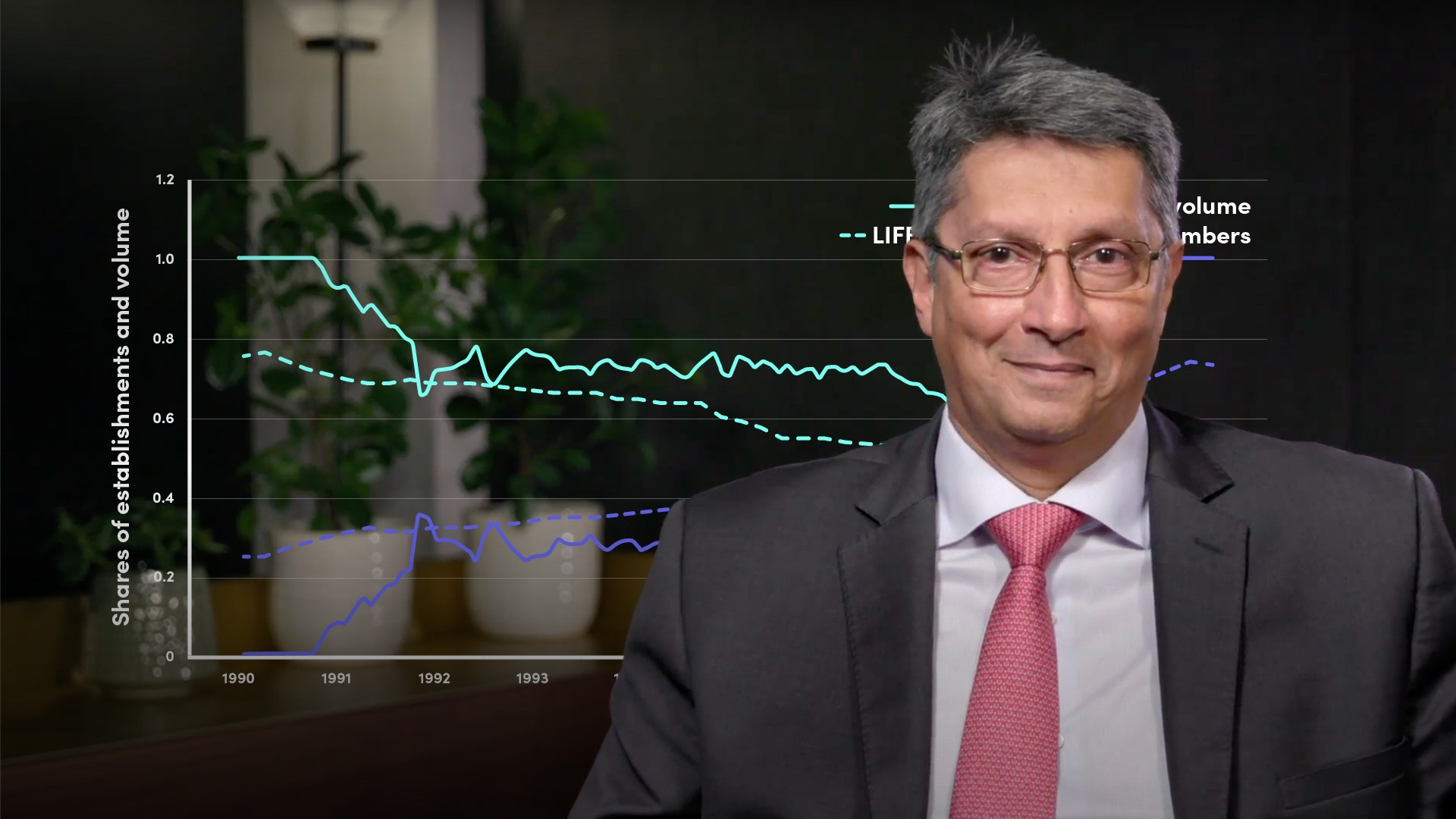04:01

## Related videosFinancial MathsFinancial MathsFinancial MathsFinancial MathsFinancial Maths### Accredited by